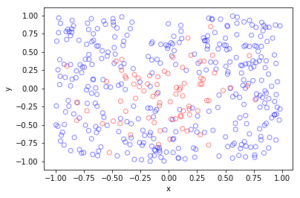## Simulating Poisson point processes faster

As an experiment, I tried to write code for simulating many realizations of a homogeneous Poisson point process in very fast fashion. My idea was to simulate all the realizations in two short steps.

In reality, the findings of this experiment and the contents of this post have little practical value, as computers are so fast at generating Poisson point processes. Still, it was an interesting task, which taught me a couple of things. And I did produce faster code.

### MATLAB

I first tried this experiment in MATLAB.

##### Vectorization

In languages like MATLAB, the trick for speed is to use vectorization, which means applying a single operation to an entire vector or matrix (or array) without using an explicit for-loop. Over the years, the people behind MATLAB has advised to use vectorization instead of for-loops, as for-loops considerably slowed down MATLAB code. (But, as as time goes by, it seems using for-loops in MATLAB doesn’t slow the code down as much as it used to.)

Simulating Poisson point processes is particularly amenable to vectorization, due to the independent nature of the point process. One can simulate the number of points in each realization for all realizations in one step. Then all the points across all realizations can also be positioned in one step. In the two-dimensional case, this results in two one-dimensional arrays (or vectors, in MATLAB parlance) for the $$x$$ and $$y$$ coordinates.  (Of course, in my code, I could have used just one two-dimensional array/vector  for the coordinates of the points, but I didn’t.)

After generating the points, the coordinates of the points need to be grouped into the different realizations and stored in appropriate data structures.

##### Data structures

The problem with storing point processes is that usually each realization has a different number of points, so more sophisticated data structures than regular arrays are needed. For MATLAB, each point process realization can be stored in a data object called a cell array.  For a structure array, it’s possible for each element (that is, structure) to be a different size, making them ideal for storing objects like point processes with randomly varying sizes.

In the case of two-dimensional point processes, two cell arrays  can be used to store the $$x$$ and $$y$$ coordinates of all the point process realizations. After randomly positioning all the points, they can be grouped into a cell array, where each cell array element represents a realization of the Poisson point process, by using the inbuilt function MATLAB mat2cell, which converts a matrix (or array) into a cell array.

Alternatively, we could use another MATLAB data object called a structure array. In MATLAB structures have fields, which can be, for example for a point process can be the locations of the points. Given cell arrays of equal size, we can convert them into a single structure array by using the inbuilt MATLAB function struct.

### Python

After successfully simulating Poisson point processes in MATLAB, I tried it in Python with the NumPy library.

##### Vectorization

I basically replicated what I did in MATLB using Python by positioning all the points in a single step. This gives two one-dimensional NumPy arrays for the $$x$$ and $$y$$ coordinates of all the point process realizations. (Again, I could have instead stored the coordinates as a single two-dimensional array, but I didn’t.)

Perhaps surprisingly, the vectorization step doesn’t speed things up much in Python with the NumPy library. This may be due to the fact that the underlying code is actually written in the C language.  That motivated me to see what methods have been implemented for simulating Poisson variables, which is the topic of the next couple posts.

##### Data structures

In Python, the data structure  known as a list is the natural choice. Similarly to cell arrays in MATLAB, two lists can be used for the $$x$$ and $$y$$ coordinates of all the points. Instead of MATLAB’s function mat2cell, I used the NumPy function numpy.split to create two lists from the two NumPy arrays containing the point coordinates.

Python does not seem to have an immediate equivalent to structure arrays in MATLAB. But in Python one can define a new data structure or class with fields, like a structure. Then one can create a list of those data structures with fields, which are called attribute references in Python.

### Code

The code in MATLAB and Python can be found here. For a comparison, I also generated the same number of point process realizations (without using vectorization) by using a trusty for-loop. The code compares the times of taken for implemented the two different approaches, labelled internally as Method A and Method B. There is a some time difference in the MATLAB code, but not much of a difference in the Python case.

I have commented out the sections that create data structures (with fields or attribute references) for storing all the point process realizations, but those sections should also work when uncommented.

## Placing a random point uniformly in a Voronoi cell

In the previous post, I discussed how Voronoi or Dirichlet tesselations are useful and how they can be calculated or estimated with many scientific programming languages by using standard libraries usually based on Qhull. The cells of such a tessellation divide the underlying space. Now I want to randomly place a single point in a uniform manner in each bounded Voronoi cell.

But why?

Well, this task arises occasionally, particularly when developing mathematical models of wireless networks, such as mobile or cellular phone networks. A former colleague of mine had to do this, which inspired me to write some MATLAB code a couple of years ago. And I’ve seen the question posed a couple of times on the web . So I thought: I can do that.

## Overview

For this problem, I see two obvious methods.

##### Simple but crude

The simplest method is to cover each Voronoi cell with a rectangle or disk. Then randomly place a point uniformly on the rectangle or disk. If it doesn’t randomly land inside the rectangle or disk, then do it again until it does. Crude, slightly inefficient, but simple.

##### Elegant but slightly tricky

A more elegant way, which I will implement, is to partition (or divide) each Voronoi cell into triangles. Then randomly choose a triangle based on its area and, finally, uniformly position a point on that triangle.

## Method

##### Partition cells into triangles

It is straightforward to divide a Voronoi cell into triangles. Each side of the cell corresponds to one side of a triangle (that is, two points). The third point of the triangle is the original point corresponding to the Voronoi cell.

##### Choose a triangle

Randomly choosing a triangle is also easy. For a given cell, number the triangles. Which random triangle is chosen is simply a discrete random variable whose probability mass function is formed from triangle areas normalized (or divided) by the total area of the Voronoi cell. In other words, the probability of randomly choosing triangle $$i$$ with area $$A_i$$ from $$m$$ triangles is simply

$$P_i=\frac{A_i}{\sum_{j=1}^m A_j}.$$

To calculate the area of the triangles, I use the shoelace formula , which for a triangle with corners labelled $$\textbf{A}$$, $$\textbf{B}$$ and $$\textbf{C}$$ gives

$$A= \frac{1}{2} |(x_{\textbf{B}}-x_{\textbf{A}})(y_{\textbf{C}}-y_{\textbf{A}})-(x_{\textbf{C}}-x_{\textbf{A}})(y_{\textbf{B}}-y_{\textbf{A}})|.$$

But you can also use Herron’s formula.

Then the random variable is sampled using the probabilities based on the triangle areas.

##### Place point on chosen triangle

Given a triangle, the final step is also easy, if you know how, which is often the case in mathematics. I have already covered this step in a previous post, but I’ll give some details here.

To position a single point uniformly in the triangle, generate two random uniform variables on the unit interval $$(0,1)$$, say $$U$$ and $$V$$. The random $$X$$ and $$Y$$ coordinates of the single point are given by the expressions:

$$X=\sqrt{U} x_{\textbf{A}}+\sqrt{U}(1-V x_{\textbf{B}})+\sqrt{U}V x_{\textbf{C}}$$

$$Y=\sqrt{U} y_{\textbf{A}}+\sqrt{U}(1-V y_{\textbf{B}})+\sqrt{U}V y_{\textbf{C}}$$

## Results

The blue points are the points of the underlying point pattern that was used to generate the Voronoi tesselation. (These points have also been arbitrarily numbered.) The red points are the random points that have been uniformly placed in all the bounded Voronoi cells.

##### MATLAB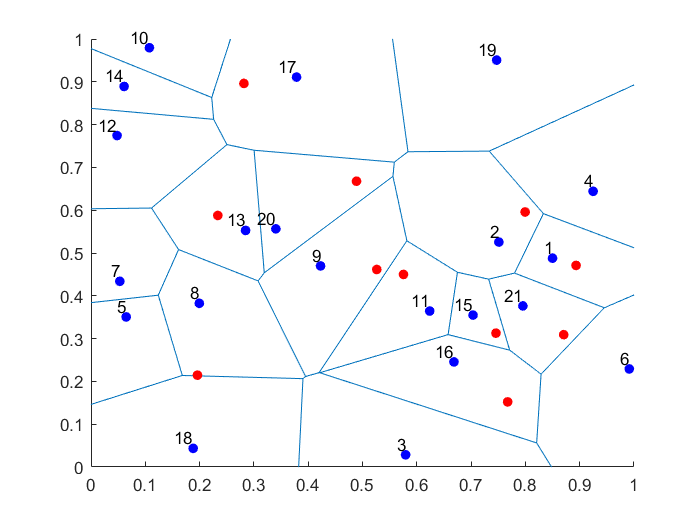##### Python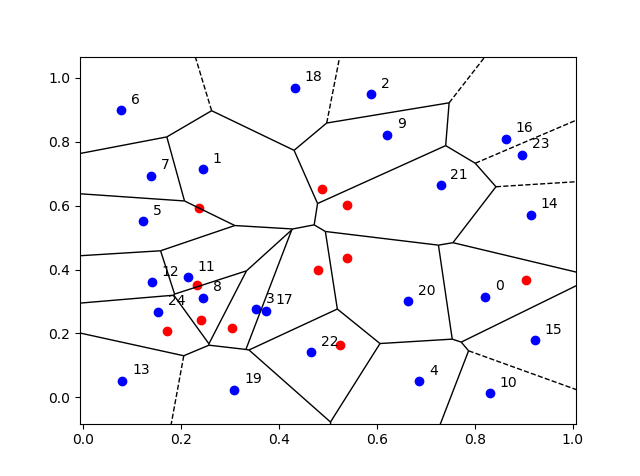## Empirical validation

We can empirically validate that the points are being placed uniformly on the bounded Voronoi cells. For a given (that is, non-random) Voronoi cell, we can repeatedly place (or sample) a random point uniformly in the cell. Increasing the number of randomly placed points, the respective averages of the $$x$$ and $$y$$ coordinates of the points will converge to the centroids (or geometric centres) of the Voronoi cell, which can be calculated with simple formulas.

## Code

The code for all my posts is located online here. For this post, the code in MATLAB and Python is here.

I have also written in MATLAB and Python the code as functions (in files funVoronoiUniform.m and funVoronoiUniform.py, respectively), so people can use it more easily. The function files are located here, where I have also included an implementation of the aforementioned empirical test involving centroids. You should be able to use those functions and for any two-dimensional point patterns.

Much has been written on Voronoi or Dirichlet tessellations, particularly when the seeds of the cells form a Poisson point process. For references, I recommend my previous post, where I say that the references in the articles on Wikipedia and MathWorld are good starting points.

In this StackExchange post, people discuss how to place a single point uniformly on a general triangle. For the formulas, the thread cites the paper Shape distributions by Osada, Funkhouser, Chazelle and Dobkin, where no proof is given.

## Voronoi tessellations

Cholera outbreaks due to public water pumps. Suburbs serviced by hospitals. Formation of crystals. Coverage regions of phone towers. We can model or approximate all these phenomena and many, many more with a geometric structure called, among other names, a Voronoi tessellation.

The main other name for this object is the Dirichlet tessellation. Historically, Dirichlet beats Voronoi, but it seems wherever I look, the name Voronoi usually wins out, suggesting an example of Stigler’s law of eponymy. A notable exception is the R library spatstat that does actually call it a Dirichlet tessellation. Wikipedia calls it Voronoi diagram. I’ve read that Descartes studied the object even earlier than Dirichlet, but Voronoi studied it in much more depth. I will call it a Voronoi tessellation.

To form a Voronoi tessellation, consider a collection of points positioned or scattered on some space, like the plane, where it’s easier to picture things, especially when using a Euclidean metric. Now for each point in the collection, consider the region surrounding the point that is closer to that point than any other point in the collection. Each region forms a cell corresponding to the point. The union of all the sets covers the underlying space.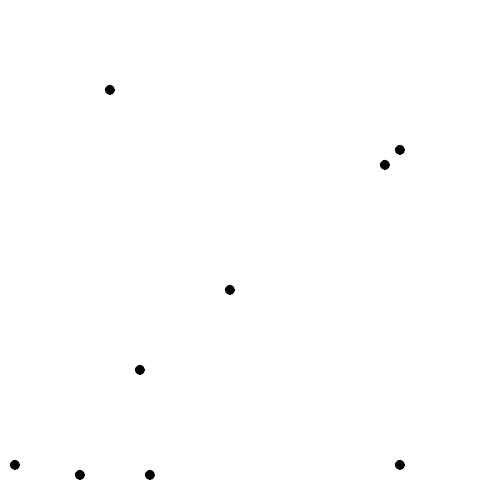The evolution of Voronoi cells, which start off as disks until they collide with each other. Source: Wikipedia.

## Everyday Voronoi tesselations

Voronoi tesselations are just not interesting mathematical objects, as they arise in everyday situations. This piece from Scientific American website explains:

Everyone uses Voronoi tessellations, even without realizing it. Individuals seeking the nearest café, urban planners determining service area for hospitals, and regional planners outlining school districts all consider Voronoi tessellations. Each café, school, or hospital is a site from which a Voronoi tessellation is generated. The cells represent ideal service areas for individual businesses, schools, or hospitals to minimize clientele transit time. Coffee drinkers, patients, or students inside a service area (that is, a cell) should live closer to their own café, hospital, or school (that is, their own cell’s site) than any other. Voronoi tessellations are ubiquitous, yet often invisible.

## Delaunay triangulation

A closely related object to the Voronoi tessellation is the Delaunay triangulation. For a given collection of points on some underlying mathematical space, a Delaunay triangulation is formed by connecting the points and creating triangles with the condition that for each point, no other point exists in the circumcircle of the corresponding triangle. (A circumcircle is a circle that passes through all three vertices of a triangle.)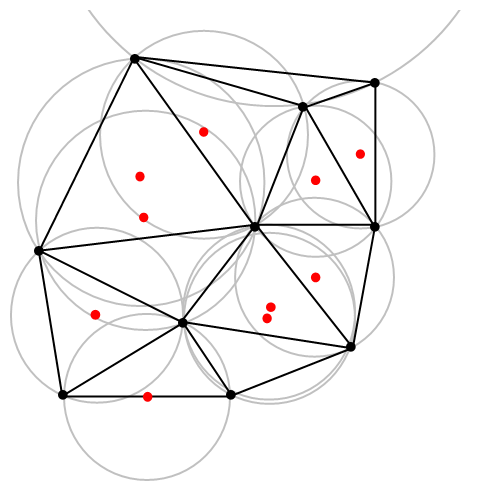An example of Delaunay triangulation with the original points in black and centrers (in red) of the corresponding circumcircles (in grey) of the Delaunay triangles. Source: Wikipedia.

The vertices of the the Delaunay triangular and Voronoi tessellation both form graphs, which turn out to be the dual graphs of each other.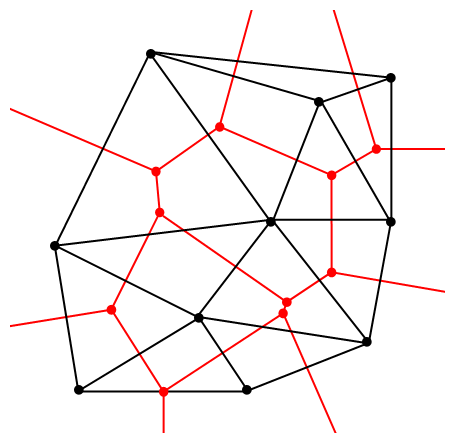A Delaunay triangulation (in black) and the corresponding Voronoi tessellation (in red) whose vertices are the centres of the circumcircles of the Delaunay triangles. Source: Wikipedia.

## Software: Qhull

Due to their applications, it’s not surprising that there exist algorithms that quickly create or estimate Voronoi tessellations. I don’t want to implement one of these algorithms from scratch, as they have already been implemented in various scientific programming languages. Many of the languages, such as MATLAB, R, and Python (SciPy) use the code from Qhull. (Note the website calls the tessellation a Voronoi diagram.)

(The Julia programming language, which I examined in in a previous post, has a Voronoi package that does not use Qhull.)

Qhull finds the Voronoi tessellation by first finding the Delaunay triangulation. If the underlying space is bounded, then all the Voronoi cells around bounded. But on unbounded space, it is possible to have unbounded cells, meaning their area (or volume) is infinite. In such cases, the algorithms sometimes place virtual points at infinity, but I don’t want to focus on such details. I will just assume Qhull does a good job.

## Code

As always, the code from all my posts is online. For this post, the MATLAB and Python code is here and here, respectively.

##### MATLAB

It is fairly straightforward to create Voronoi tessellations in MATLAB. You can just use the function voronoi, which is only for two-dimensional tessellations. (Note: the MATLAB website says the behaviour of the function voronoi has changed, so that may cause problems when using different versions of MATLAB.) The function requires two inputs as vectors, say, x and y, corresponding to the Cartesian (or $$x$$ and $$y$$) coordinates of the points. If you just run the voronoi command, it will create and plot a Voronoi tessellation (or Voronoi diagram, as MATLAB calls it). But the MATLAB website also describes how to plot the tessellation manually.

For d-dimensional tessellations, there is the function voronoin, which requires a single input. The single output consists of combining $$d$$ column vectors for the Cartesian coordinates. For example, given the column vectors x, y and z, then the input is [x, y, z].

If you give the functions voronoi or voronoin output arguments, then the tessellation is not plotted and instead two data structures, say, v and c are created for describing the vertices of the tessellation. I generally use voronoi for plotting, but I use voronoin (and not voronoi) for generating vertex data, so I will focus on the outputs of voronoin.

For voronoin, the first (output) data structure v is simply an two-dimensional array array that contain the Cartesian coordinates of every vertex in the Voronoi tessellation. The second (output) data structure c is a cell array describing the vertices of each Voronoi cell (it has to be a cell array, as opposed to a regular array, as the cells have varying number of vertices). Each entry of the cell array contains a one-dimensional array with array indices corresponding to the $$x$$ and $$y$$ coordinates.

The code checks which Voronoi cells are unbounded by seeing if they have vertices at infinity, which corresponds to a $$1$$ in the index arrays (stored in the structure array c).

##### Python

To create the Voronoi tessellation, use the SciPy (Spatial) function Voronoi. This function does $$d$$-dimensional tessellations. For the two-dimensional setting, you need to input the $$x$$ and $$y$$ coordinates as a single array of dimensions $$2 \times n$$, where $$n$$ is the number of points in the collection. In my code, I start off with two one-dimensional arrays for the Cartesian coordinates, but then I combined them into a single array by using the function numpy.stack with the function argument axis =1.

I would argue that the Voronoi function in SciPy is not as intuitive to use as the MATLAB version. For example, one thing I found a bit tricky, at first, is that the cells and the points have a different sets of numbering (that is, they are indexed differently). (And I am not the only one that was caught by this.) You must use the attribute called point_region to access a cell number (or index) from a point number (or index). In my code attribute is accessed and then called it indexP2C, which is an integer array with cell indices. Apart from that, the function Voronoi worked well.

To plot the Voronoi tessellation, use the SciPy function voronoi_plot_2d, which allows for various plotting options, but it does require Matplotlib. The input is the data object created by the function Voronoi.

## Results

I’ve plotted the results for a single realization of a Poisson point process. I’ve also plotted the indices of the points. Recall that the indexing in Python and MATLAB start respectively at zero and one.

##### MATLAB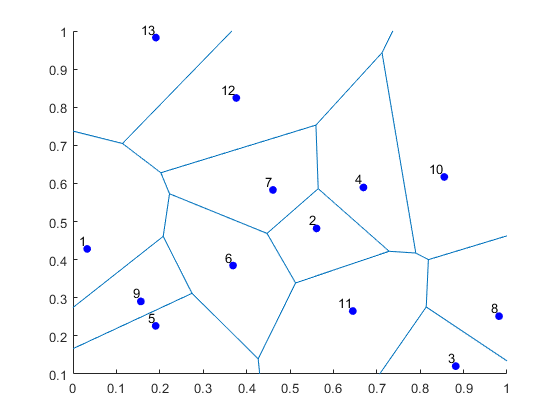##### Python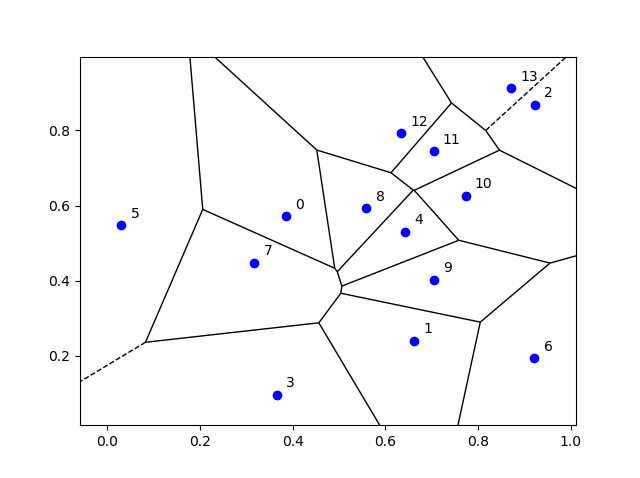## Voronoi animations

I took the animation of evolving Voronoi cells, which appears in the introduction, from Wikipedia. The creator generated it in MATLAB and also posted the code online. The code is long, and I wouldn’t even dare to try to reproduce it, but I am glad someone else wrote it.

Such animations exist also for other metrics. For example, the Manhattan metric (or taxi cab or city block metric) gives the following animations, where the growing disks have been replaced with squares.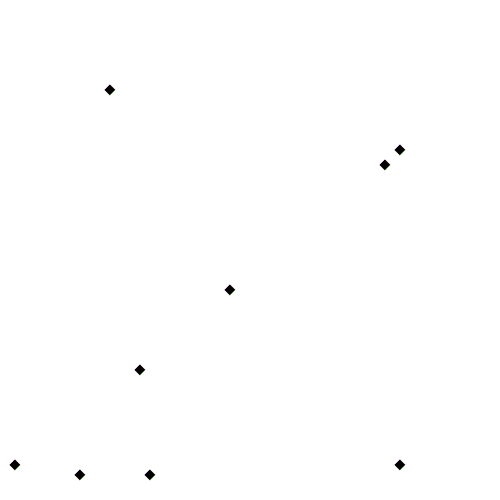This Wikipedia user page has animations under other metrics on Euclidean space.

This post also animations of Voronoi tessellations when the points move.

There is a lot of literature on Voronoi or Dirichlet tessellations, particularly when the seeds of the cells form a Poisson point process. The references in the articles on Wikipedia and MathWorld are good starting points.

Here is a good post on Voronoi tesselations. Here is a non-mathematical article published in the Irish Times.

For the more mathematically inclined, there is also the monograph Lectures on random Voronoi tessellations by Møller.

## Simulating a Cox point process based on a Poisson line process

In the previous post, I described how to simulate a Poisson line process, which in turn was done by using insight from an earlier post on the Bertrand paradox.

Now, given a Poisson line process, for each line, if we generate an independent one-dimensional Poisson point point process on each line, then we obtain an example of a Cox point process. Cox point processes are also known as doubly stochastic Poisson point processes. On the topic of names, Guttorp and Thorarinsdottir argue that it should be called the Quenouille point process, as Maurice Quenouille introduced an example of it before Sir David Cox, but I opt for the more common name.

## Cox point proceesses

A Cox point process is a generalization of a Poisson point process. It is created by first considering a non-negative random measure, sometimes called a driving measure. Then a Poisson point process, which is independent of the random driving measure, is generated by using the random measure as its intensity or mean measure.

The driving measure of a Cox point process can be, for example, a non-negative random variable or field multiplied by a Lebesgue measure. In our case, the random measure is the underlying Poisson line process coupled with the Lebesgue measure on the line (that is, length).

Cox processes form a very large and general family of point processes, which exhibit clustering. In previous posts, I have covered two special cases of Cox point processes: the Matérn and Thomas cluster point processes. These are, more specifically, examples of a Neyman-Scott point process, which is a special case of a shot noise Cox point process. These two point processes are fairly easy to simulate, but that’s not the case for Cox point processes in general. Some are considerably easier than others.

## Motivation

I will focus on simulating the Cox point process formed from a Poisson line process with homogeneous Poisson point processes. I do this for two reasons. First, it’s easy to simulate, given we can simulate a Poisson line process. Second, it has been used and studied recently in the mathematics and engineering literature for investigating wireless communication networks in cities, where the streets correspond to Poisson lines; for example, see these two preprints:

Incidentally, I don’t know what to call this particular Cox point process. A Cox line-point process? A Cox-Poisson line-point process? But it doesn’t matter for simulation purposes.

## Method

We will simulate the Cox (-Poisson line-) point process on a disk. Why a disk? I suggest reading the previous posts on the Poisson line process the Bertrand paradox for why the disk is a natural simulation window for line processes.

Provided we can simulate a Poisson line process, the simulation method is quite straightforward, as I have essentially already described it.

##### Line process

First simulate a Poisson line process on a disk. We recall that for each line of the line process, we need to generate two independent random variables $$\Theta$$ and $$P$$ describing the position of the line. The first random variable $$\Theta$$ gives the line orientation, and it is a uniform random variable on the interval $$(0,2\pi)$$.

The second random variables $$P$$ gives the distance from the origin to the disk edge, and it is a uniform random variable on the interval $$(0,r)$$, where $$r$$ is the radius of the disk. The distance from the point $$(\Theta, P)$$ to the disk edge (that is, the circle) along the chord is:

$$Q=\sqrt{r^2-P^2}.$$

The endpoints of the chord (that is, the points on the disk edge) are then:

Point 1: $$X_1=P \cos \Theta+ Q\sin \Theta$$, $$Y_1= P \sin \Theta- Q\cos \Theta$$,

Point 2: $$X_2=P \cos \Theta- Q\sin \Theta$$, $$Y_2= P \sin \Theta+Q \cos \Theta$$.

The length of the line segment is $$2 Q$$. We can say this random line is described by the point $$(\Theta,P)$$.

##### One-dimensional Poisson point process

For each line (segment) in the line process, simulate a one-dimensional Poisson point process on it. Although I have never discussed how to simulate a one-dimensional (homogeneous) Poisson point process, it’s essentially one dimension less than simulating a homogeneous Poisson point process on a rectangle.

More specifically, given a line segment $$(\Theta,P)=(\theta,p)$$, you simulate a homogeneous Poisson point process with intensity $$\mu$$ on a line segment with length $$2 q$$, where $$q=\sqrt{r^2-p^2}$$. (I am now using lowercase letters to stress that the line is no longer random.) To simulate the homogeneous Poisson point process, you generate a Poisson random variable with parameter $$2 \mu q$$.

Now you need to place the points uniformly on the line segment. To do this, consider a single point on a single line. For this point, generate a single uniform variable $$U$$ on the interval $$(-1,1)$$. The tricky part is now getting the Cartesian coordinates right. But the above expressions for the endpoints suggest that the single random point has the Cartesian coordinates:

$$x=p \cos \theta+ U q\sin \theta$$, $$y=p \sin \theta- U q\cos \theta$$.

The two extreme cases of the uniform random variable $$U$$ (that is, $$U=-1$$ and $$U=1$$) correspond to the two endpoints of the line segment. We recall that $$Q$$ is the distance from the midpoint of the line segment to the disk edge along the line segment, so it makes sense that we want to vary this distance uniformly in order to uniformly place a point on the line segment. This uniform placement step is done for all the points of the homogeneous Point process on that line segment.

You repeat this procedure for every line segment. And that’s it: a Cox point process built upon a Poisson line process.

## Results

##### MATLAB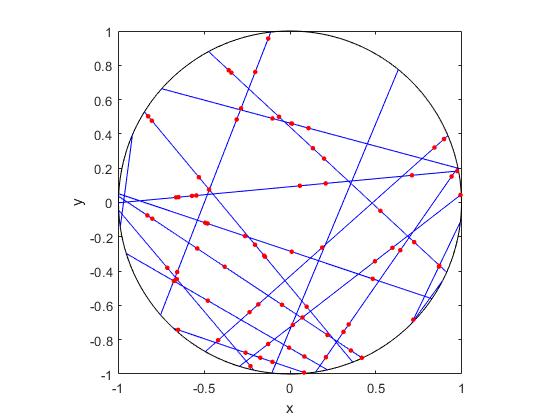##### R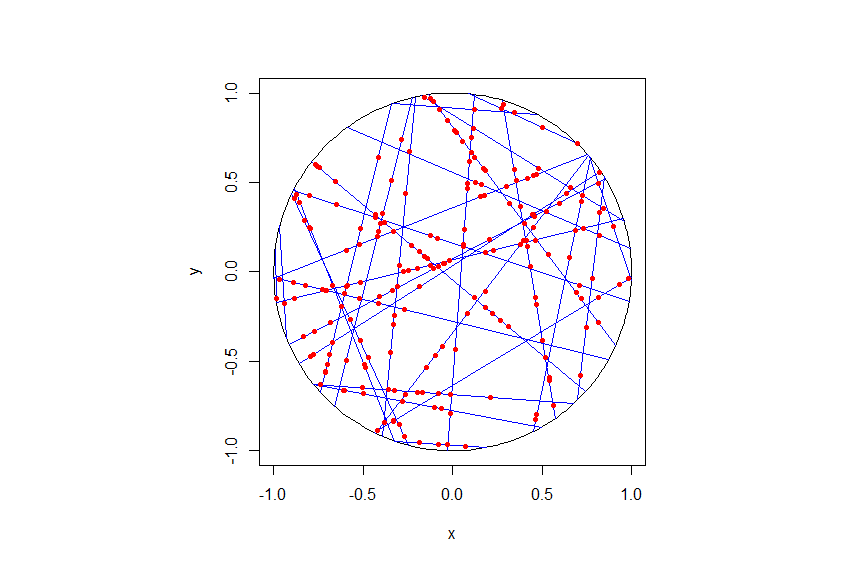##### Python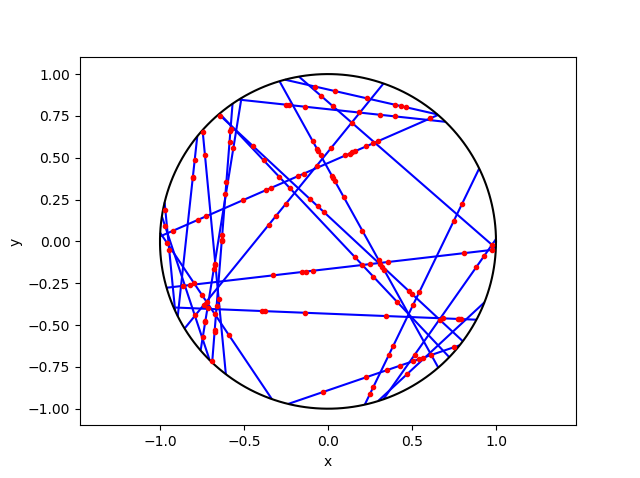## Code

As always, the code from all my posts is online. For this post, I have written the code in MATLAB, R and Python.

For the first step, the reading material is basically the same as that for the Poisson line process, which overlaps with that of the Bertrand paradox. For the one-dimensional Poisson point process, we can use the reading material on the homogeneous Poisson point process on a rectangle.

For general Cox point processes, I recommend starting with the following: Chapter 6 in the monograph Poisson processes by Kingman; Chapter 5 in Statistical Inference and Simulation for Spatial Point Processes by Møller and Waagepetersen; and Section 5.2 in Stochastic Geometry and its Applications by Chiu, Stoyan, Kendall and Mecke. For a much more mathematical treatment, see Chapter 13 in Lectures on the Poisson Process by Last and Penrose.

For this particularly Cox point process, see the two aforementioned preprints, located here and here.

## Simulating a Poisson line process

Instead of points, we can consider other objects randomly scattered on some underlying mathematical space. If we take a Poisson point process, then we can use (infinitely long) straight lines instead of points, giving a Poisson line process. Researchers have studied and used this random object to model physical phenomena. In this post I’ll cover how to simulate a homogeneous Poisson line process in MATLAB, R and Python. The code which can be downloaded from here

## Overview

For simulating a Poisson line process, the key question is how to randomly position the lines.  This is related to a classic problem in probability theory called the Bertrand paradox.  I discussed this illustration in probability in a previous post, where I also included code for simulating it. I highly recommend reading that post first.

The Bertrand paradox involves three methods for positioning random lines. We use Method 2 to achieve a uniform positioning of lines, meaning the number of lines and orientation of lines is statistically uniform. Then it also makes sense to use this method for simulating a homogeneous (or uniform) Poisson line process.

We can interpret a line process as a point process. For a line process on the plane $$\textbf{R}^2$$, it can be described by a point process on $$(0,\infty)\times (0,2\pi)$$, which is an an infinitely long cylinder. In other words, the Poisson line process can be described as a Poisson point process.

For simulating a Poisson line process, it turns out the disk is the most natural setting. (Again, this goes back to the Bertrand paradox.) More specifically, how the (infinitely long) lines intersect a disk of a fixed radius $$r>0$$. The problem of simulating a Poisson line process reduces to randomly placing chords in a disk. For other simulation windows in the plane, we can always bound any non-circular region with a sufficiently large disk.

## Steps

##### Number of lines

Of course, with all things Poisson, the number of lines will be  a Poisson random variable, but what will its parameter be? This homogeneous (or uniform) Poisson line process forms a one-dimensional homogeneous (or uniform) Poisson point process around the edge of the disk with a circumference $$2 \pi r$$. Then the number of lines is simply a Poisson variable with parameter $$\lambda 2 \pi r$$.

##### Locations of points

To position a single line uniformly in a disk, we need to generate two uniform random variables. One random variable gives the angle describing orientation of the line, so it’s a uniform random variable $$\Theta$$ on the interval $$(0,2\pi)$$.

The other random variable gives the distance from the origin to the disk edge, meaning it’s a uniform random variable $$P$$ on the interval $$(0,r)$$, where $$r$$ is the radius of the disk.  The random radius and its perpendicular chord create a right-angle triangle.  The distance from the point $$(\Theta, P)$$ to the disk edge (that is, the circle) along the chord is:

$$Q=\sqrt{r^2-P^2}.$$

The endpoints of the chord (that is, the points on the disk edge) are then:

Point 1: $$X_1=P \cos \Theta+ Q\sin \Theta$$, $$Y_1= P \sin \Theta- Q\cos \Theta$$,

Point 2: $$X_2=P \cos \Theta- Q\sin \Theta$$, $$Y_2= P \sin \Theta+Q \cos \Theta$$.

## Code

I have implemented the simulation procedure in MATLAB, R and Python, which, as usual, are all very similar. I haven’t put my code here, because the software behind my website keeps mangling it.  As always, I have uploaded my code to a repository; for this post, it’s in this directory.

I have written the code in R, but I wouldn’t use it in general. That’s because if you’re using R, then, as I have said before, I strongly recommend using the powerful spatial statistics library spatstat. For a simulating Poisson line process, there’s the function rpoisline.

The chief author of spatstat, Adrian Baddeley, has written various lectures and books on the related topics of point processes, spatial statistics, and geometric probability. In this post, he answered why the angular coordinates have to be chosen uniformly.

### Results

MATLAB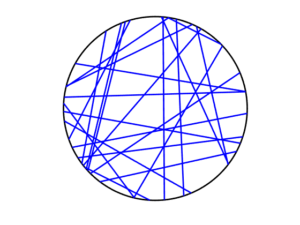R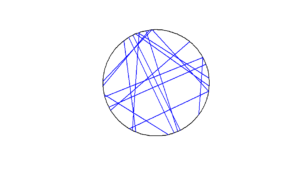Python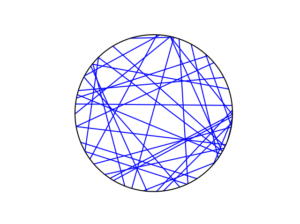To read about the Poisson line process, it’s best to start with the Bertrand problem, which is covered in many works on geometric probability and related fields. A good and recent introduction is given by Calka in (Section 1.3) of the lectures titled Stochastic Geometry: Modern Research Frontiers, which were edited by Coupier and published by Springer.  Also see, for example, problem 1.2 in Geometrical Probability by Kendall and Moran or page 44 in Geometric Probability by Solomon.

For the Poisson line process, I highly recommend Section 7.2 in the monograph Poisson processes by Kingman. Also see Example 8.2 in the standard textbook Stochastic Geometry and its Applications by Chiu, Stoyan, Kendall and Mecke. The Poisson line process book also appears in Exercise 5.2 in the book Stochastic Simulation – Algorithms and Analysis by  Asmussen and Glynn.

For online resources, this set of lectures by Christian Lantuéjoul
covers the Poisson line process. Wilfrid Kendall covers the Poisson line process in this talk in relation to so-called Poisson cities.

Mathematical paradoxes are results or observations in mathematics that are (seemingly) conflicting, unintuitive, incomprehensible, or just plain bizarre. They come in different flavours, such as those that play with notions of infinity, which means they often make little or no sense in a physical world. Other paradoxes, particularly those in probability, serve as a lesson that the problem needs to be posed in a precise manner. The Bertrand paradox is one of these.

Joseph Bertrand posed the original problem in his 1889 book Calcul des probabilités, which is available online (albeit in French). It’s a great illustrative problem involving simple probability and geometry, so it often appears in literature on the (closely related) mathematical fields of geometric probability and integral geometry.

Based on constructing a random chord in a circle, the paradox involves a single mathematical problem with three reasonable but different solutions. It’s less a paradox and more a cautionary tale. It’s really asking the question: What do you mean by random?

Consequently, over the years the Bertrand paradox has inspired debate, with papers arguing what the true solution is. I recently discovered it has even inspired some passionate remarks on the internet; read the comments at www.bertrands-paradox.com.

But I am less interested in the different interpretations or philosophies of the problem. Rather, I want to simulate the three solutions. This is not very difficult, provided some trigonometry and knowledge from a previous post, where I describe how to simulate a (homogeneous) Poisson point process on the disk.

I won’t try to give a thorough analysis of the solutions, as there are much better websites doing that. For example, this MIT website gives a colourful explanation with pizza and fire-breathing monsters. The Wikipedia article also gives a detailed though less creative explanation for the three solutions.

My final code in MATLAB, R and Python code is located here.

### The Problem

Bertrand considered a circle with an equilateral triangle inscribed it. If a chord in the circle is randomly chosen, what is the probability that the chord is longer than a side of the equilateral triangle?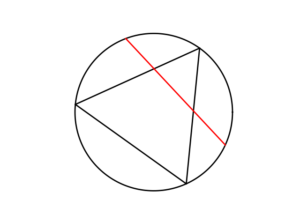### The Solution(s)

Bertrand argued that there are three natural but different methods to randomly choose a chord, giving three distinct answers. (Of course, there are other methods, but these are arguably not the natural ones that first come to mind.)

##### Method 1: Random endpoints

On the circumference of the circle two points are randomly (that is, uniformly and independently) chosen, which are then used as the two endpoints of the chord.

The probability of this random chord being longer than a side of the triangle is one third.

A radius of the circle is randomly chosen (so the angle is chosen uniformly), then a point is randomly (also uniformly) chosen along the radius, and then a chord is constructed at this point so it is perpendicular to the radius.

The probability of this random chord being longer than a side of the triangle is one half.

##### Method 3: Random midpoint

A point is randomly (so uniformly) chosen in the circle, which is used as the midpoint of the chord, and the chord is randomly (also uniformly) rotated.

The probability of this random chord being longer than a side of the triangle is one quarter.

### Simulation

All three answers involve randomly and independently sampling two random variables, and then doing some simple trigonometry. The setting naturally inspires the use of polar coordinates. I assume the circle has a radius $$r$$ and a centre at the point origin $$o$$. I’ll number the end points one and two.

In all three solutions we need to generate uniform random variables on the interval $$(0, 2\pi)$$ to simulate random angles. I have already done this a couple of times in previous posts such as this one.

##### Method 1: Random endpoints

This is probably the most straightforward solution to simulate. We just need to simulate two uniform random variables $$\Theta_1$$ and $$\Theta_2$$ on the interval $$(0, 2\pi)$$ to describe the angles of the two points.

The end points of the chord (in Cartesian coordinates) are then simply:

Point 1: $$X_1=r \cos \Theta_1$$, $$Y_1=r \sin \Theta_1$$,

Point 2: $$X_2=r \cos \Theta_2$$, $$Y_2=r \sin \Theta_2$$.

This method also involves generating two uniform random variables. One random variable $$\Theta$$ is for the angle, while the other $$P$$ is the random radius, which means generating the random variable $$P$$ on the interval $$(0, r)$$.

I won’t go into the trigonometry, but the random radius and its perpendicular chord create a right-angle triangle. The distance from the point $$(\Theta, P)$$ to the circle along the chord is:

$$Q=\sqrt{r^2-P^2}.$$

The endpoints of the chord are then:

Point 1: $$X_1=P \cos \Theta+ Q\sin \Theta$$, $$Y_1= P \sin \Theta- Q\cos \Theta$$,

Point 2: $$X_2=P \cos \Theta- Q\sin \Theta$$, $$Y_2= P \sin \Theta+Q \cos \Theta$$.

Take note of the signs in these expressions.

##### Method 3: Random midpoint

This method requires placing a point uniformly on a disk, which is also done when simulating a homogeneous Poisson point process on a disk, and requires two random variables $$\Theta’$$ and $$P’$$. Again, the angular random variable $$\Theta’$$ is uniform.

The other random variable $$P’$$ is not uniform. For $$P’$$, we generate a random uniform variable on the unit interval $$(0,1)$$, and then we take the square root of it. We then multiply it by the radius, generating a random variable between $$0$$ and $$r$$. (We must take the square root because the area element of a sector is proportional to the radius squared, and not the radius.) The distribution of this random variable is an example of the triangular distribution.

The same trigonometry from Method 2 applies here, which gives the endpoints of the chord as:

Point 1: $$X_1=P’ \cos \Theta’+ Q’\sin \Theta’$$, $$Y_1= P’ \sin \Theta’- Q’\cos \Theta’$$,

Point 2: $$X_2=P’\cos \Theta’- Q’\sin \Theta’$$, $$Y_2= P’\sin \Theta’+Q’ \cos \Theta’$$,

where $$Q’:=\sqrt{r^2-{P’}^2}$$. Again, take note of the signs in these expressions.

### Results

To illustrate how the three solutions are different, I’ve plotted a hundred random line segments and their midpoints side by side. Similar plots are in the Wikipedia article.

#####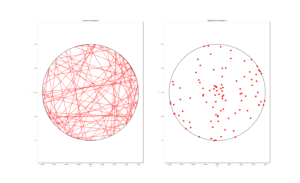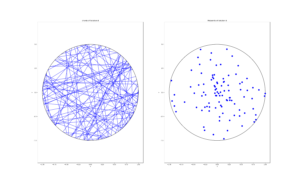##### Method 3: Random midpoint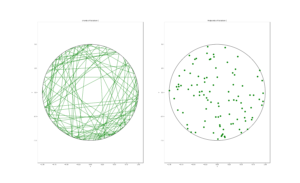### Conclusion

For the chord midpoints, we know and can see that Method 3 gives uniform points, while Method 2 has a concentration of midpoints around the circle centre. Method 1 gives results that seem to somewhere between Method 2 and 3 in terms of clustering around the circle centre.

For the chords, we see that Method 3 results in fewer chords passing through the circle centre. Methods 1 and 2 seem to give a similar number of lines passing through this central region.

It’s perhaps hard to see, but it can be shown that Method 2 gives the most uniform results. By this, I mean that the number of lines and their orientations statistically do not vary in different regions of the circle.

We can now position random lines in uniform manner. All we need now is a Poisson number of lines to generate something known as a Poisson line process, which will be the focus of the next post.

I’ve already mentioned that there are some good websites on the topic of the Bertrand paradox. For example:

web.mit.edu/tee/www/bertrand

www.cut-the-knot.org/bertrand.shtml

mathworld.wolfram.com/BertrandsProblem.html

Various authors have mentioned or discussed the Bertrand paradox in books on the related subjects of geometric probability, integral geometry and stochastic geometry. A good and recent introduction is given by Calka in Section 1.3 of the published lectures Stochastic Geometry: Modern Research Frontiers.

Other classic books that cover the topic including, for example, see Problem 1.2 in Geometrical Probability by Kendall and Moran. (Despite Maurice G. Kendall writing a book on geometric probability, he was not related to stochastic geometry pioneer David G Kendall.) It’s also discussed on page 44 in Geometric Probability by Solomon. For a book that involves more advance knowledge of geometry and (abstract) algebra, see Chapter 3 in Integral Geometry and Geometric Probability by Santaló.

The Bertrand paradox is also in The Pleasures of Probability by Isaac. It’s covered in a non-mathematical way in the book Paradoxes from A to Z by Clark. Edwin Jaynes studied the problem and proposed a solution in a somewhat famous 1973 paper, titled The Well-Posed Problem.

The original problem can be read in French in Bertrand’s work, which is available online here or here (starting at the bottom of page 4).

### Code

The MATLAB, R and Python code can be found here. In the code, I have labelled the methods A, B and C instead 1, 2 and 3.

## Testing the Julia language with point process simulations

I started writing these posts (or blog entries) about a year ago. In my first post I remarked how I wanted to learn to write stochastic simulations in a new language. Well, I found one. It’s called Julia. Here’s my code. And here are my thoughts.

### Overview

For scientific programming, the Julia language has arisen as a new contender. Originally started in 2012, its founders and developers have (very) high aspirations, wanting the language to be powerful and accessible, while still having run speeds comparable to C. There’s been excitement about it, and even a Nobel Laureate in economics, Thomas Sargent, has endorsed it. He co-founded the QuantEcon project, whose website has this handy guide or cheat sheet for commands between MATLAB, Python and Julia.

That guide suggests that Julia’s main syntax inspiration comes from MATLAB. But perhaps its closest (and greatest) competitor in scientific programming languages is Python, which has become a standard language used in scientific programming, particularly in machine learning. Another competitor is the statistics language R, which is popular for data science. But R is not renown for its speed.

As an aside, machine learning is closely related to what many call data science. I consider the two disciplines as largely overlapping with statistics, where their respective emphases are on theory and practice. In these fields, often the languages Python and R are used. There are various websites discussing which language is better, such as this one, which in turn is based on this one. In general, it appears that computer scientists and statisticians respectively prefer using Python and R.

Returning to the Julia language, given its young age, the language is still very much evolving, but I managed to find suitable Julia functions for stochastic simulations. I thought I would try it out by simulating some point processes, which I have done several times before. I successfully ran all my code with Julia Version 1.0.3.

In short, I managed to replicate in (or even translate to) Julia the code that I presented in the following posts:

Simulating a homogeneous Poisson point process on a rectangle

Simulating a Poisson point process on a disk

Simulating a Poisson point process on a triangle

Simulating an inhomogeneous Poisson point process

Simulating a Matérn cluster point process

Simulating a Thomas cluster point process

The Julia code, like all the code I present here, can be found on my Github repository, which for this post is located here.

### Basics

##### Language type and syntax

The Wikipedia article on Julia says:

Julia is a high-level general-purpose dynamic programming language designed for high-performance numerical analysis and computational science.

Scientific programming languages like the popular three MATLAB, R and Python, are interpreted languages. But the people behind Julia say:

it is a flexible dynamic language, appropriate for scientific and numerical computing, with performance comparable to traditional statically-typed languages.

Because Julia’s compiler is different from the interpreters used for languages like Python or R, you may find that Julia’s performance is unintuitive at first.

I already remarked that Julia’s syntax is clearly inspired by MATLAB, as one can see in this guide for MATLAB, Python and Julia. But there are key differences. For example, to access an array entry in Julia, you use square brackets (like in most programming languages), whereas parentheses are used in MATLAB.

##### Packages

Julia requires you to install certain packages or libraries, like most languages. For random simulations and plots, you have to install the Julia packages Distributions and Plots, which is done by running the code.

Pkg.add("Distributions");
Pkg.add("Plots");

After doing that, it’s best to restart Julia. These packages are loaded with the using command:

Using Distributions;
Using Plots;

Also, the first time it takes a while to run any code using those newly installed packages.

I should stress that there are different plotting libraries, but Plots, which contains many plotting libraries, is the only one I could get working. Another is PlotPy, which uses the Python library. As a beginner, it seems to me that the Julia community has not focused too much on developing new plotting functions, and has instead leveraged pre-existing libraries.

For standard scientific and statistical programming, you will usually also need the packages LinearAlgebra and Statistics.

##### Data types

Unlike MATLAB or R, Julia is a language that has different data types for numbers, such as integers and floating-point numbers (or floats). This puts Julia in agreement with the clear majority of languages, making it nothing new for most programmers. This is not a criticism of the language, but this can be troublesome if you’ve grown lazy after years of using MATLAB and R.

##### Simulating random variables

In MATLAB, R and Python, we just need to call a function for simulating uniform, Poisson, and other random variables. There’s usually a function for each type of random variable (or probability distribution).

Julia does simulation of random objects in a more, let’s say, object-oriented way (but I’m told, it’s not an object-oriented language). The probability distributions of random variables are objects, which are created and then sent to a general function for random generation. For example, here’s the code for simulating a Poisson variable with mean $$\mu=10$$.

mu=10;
distPoisson=Poisson(mu);
numbPoisson=rand(distPoisson);

Similarly, here’s how to simulate a normal variable with mean $$\mu=10$$ and standard deviation $$\sigma=1$$.

sigma=1;
distNormal=Normal(mu,sigma);
numbNormal=rand(distNormal);

Of course the last two lines can be collapsed into one.

mu=10;
sigma=1;
numbNormal=rand(Normal(mu,sigma));

But if you just want to create standard uniform variables on the interval (0,1), then the code is like that in MATLAB. For example, this code creates a $$4\times3$$ matrix (or array) $$X$$ whose entries are simulation outcomes of independent uniform random variables:

X=rand(4,3);

The resulting matrix $$X$$ is a Float 64 array.

##### Arrays

The indexing of arrays in Julia starts at one, like MATLAB and R. When you apply a function to an array, you generally need to use the dot notation. For example, if I try to run the code:

Y=sqrt(rand(10,1)); #This line will result in an error.

then on my machine (with Julia Version 1.0.3) I get the error:

ERROR: DimensionMismatch(“matrix is not square: dimensions are (10, 1)”)

But this code works:

Y=sqrt.(rand(10,1));

Also, adding scalars to arrays can catch you in Julia, as you also often need to use the dot notation. This code:

Y=sqrt.(rand(10,1));
Z=Y+1; #This line will result in an error.

gives the error:

ERROR: MethodError: no method matching +(::Array{Float64,2}, ::Int64)

This is fixed by adding a dot:

Y=sqrt.(rand(10,1));
Z=Y.+1; #This line will work.

Note the dot has to be on the left hand side. I ended up just using dot notation every time to be safe.

Other traps exist. For example, with indexing, you need to convert floats to integers if you want to use them as indices.

##### Repeating array elements

There used to be a Julia function called repmat, like the one in MATLAB , but it was merged with a function called repeat. I used such repeating operations to avoid explicit for-loops, which is generally advised in languages like MATLAB and R. For example, I used the repelem function in MATLAB to simulate Matérn and Thomas cluster point processes. To do this in Julia, I had to use this nested construction:

y=vcat(fill.(x, n)...);

This line means that the first value in $$x$$ is repeated $$n$$ times, where $$n$$ is the first entry of $$n$$ (as indexing in Julia starts at one), then the second value of $$x$$ is repeated $$n$$ times, and so on. For example, the vectors $$x=[7,4,9]$$ and $$n=[2,1,3]$$, the answer is $$y=[7,7,4,9,9,9]$$.

To do this in Julia, the construction is not so bad, if you know how, but it’s not entirely obvious. In MATLAB I use this:

y=repelem(x,n);

Similarly in Python:

y=np.repeat(x,n);
##### Different versions of Julia

I found that certain code would work (or not work) and then later the same code would not work (or would work) on machines with different versions of Julia, demonstrating how the language is still being developed. More specifically, I ran code on Julia Version 1.0.3 (Date 2018-12-18) and Julia Version 0.6.4 (Date: 2018-07-09). (Note how there’s only a few months difference in the dates of the two versions.)

Consider the code with the errors (due to the lack of dot operator) in the previous section. The errors occurred on one machine with Julia Version 1.0.3, but the errors didn’t occur on another machine with the older Julia Version 0.6.4. For a specific example, the code:

Y=sqrt.(rand(10,1));
Z=Y+1; #This line will not result in an error on Version 0.6.4.

gives no error with Julia Version 0.6.4, while I have already discussed how it gives an error with Julia Version 1.0.3.

For another example, I copied from this MATLAB-Python-Julia guide the following command:

A = Diagonal([1,2,3]); #This line will (sometimes?) result in an error.

It runs on machine with Julia Version 0.6.4 with no problems. But running it on the machine with Julia Version 1.0.3 gives the error:

ERROR: UndefVarError: Diagonal not defined

That’s because I have not used the LinearAlgebra package. Fixing this, the following code:

using LinearAlgebra; #Package needed for Diagonal command.
A = Diagonal([1,2,3]); #This line should now work.

gives no error with Julia Version 1.0.3.

If you have the time and energy, you can search the internet and find online forums where the Julia developers have discussed why they have changed something, rendering certain code unworkable with the latest versions of Julia.

##### Optimization

It seems that performing optimization on functions is done with the Optim package.

Pkg.add("Optim");

But some functions need the Linesearches package, so it’s best to install that as well.

Pkg.add("Linesearches");

Despite those two optimization packages, I ended up using yet another package called BlackBoxOptim.

Pkg.add("BlackBoxOptim");

In this package, I used a function called bboptimize. This is the first optimziation function that I managed to get working. I do not know how it compares to the functions in the Optim and Linesearches packages.

In a previous post, I used optimization functions to simulate a inhomogeneous or nonhomogeneous Poisson point process on a rectangle. I’ve also written Julia code for this simulation, which is found below. I used bboptimize, but I had some problems when I initially set the search regions to integers, which the package did not like, as the values need to be floats. That’s why I multiple the rectangle dimensions by $$1.0$$ in the following code:

boundSearch=[(1.0xMin,1.0xMax), (1.0yMin, 1.0yMax)]; #bounds for search box
#WARNING: Values of boundSearch cannot be integers!
resultsOpt=bboptimize(fun_Neg;SearchRange = boundSearch);
lambdaNegMin=best_fitness(resultsOpt); #retrieve minimum value found by bboptimize

## Conclusion

In this brief experiment, I found the language Julia good for doing stochastic simulations, but too tricky for doing simple things like plotting. Also, depending on the version of Julia, sometimes my code would work and sometimes it wouldn’t. No doubt things will get better with time.

As I said, Julia is still very much an ongoing project. Here’s a couple of links that helped me learn the basics.

https://en.wikibooks.org/wiki/Introducing_Julia/Arrays_and_tuples

https://voxeu.org/content/which-numerical-computing-language-best-julia-matlab-python-or-r

Julia, Matlab, and C

https://modelingguru.nasa.gov/docs/DOC-2676

## Code

I’ve only posted here code for some of simulations, but the rest of the code is available on my GitHub repository located here. You can see how the code is comparable to that of MATLAB.

##### Poisson point process on a rectangle

using Distributions #for random simulations
using Plots #for plotting

#Simulation window parameters
xMin=0;xMax=1;
yMin=0;yMax=1;
xDelta=xMax-xMin;yDelta=yMax-yMin; #rectangle dimensions
areaTotal=xDelta*yDelta;

#Point process parameters
lambda=100; #intensity (ie mean density) of the Poisson process

#Simulate Poisson point process
numbPoints=rand(Poisson(areaTotal*lambda)); #Poisson number of points
xx=xDelta*rand(numbPoints,1).+xMin;#x coordinates of Poisson points
yy=yDelta*(rand(numbPoints,1)).+yMin;#y coordinates of Poisson points

#Plotting
plot1=scatter(xx,yy,xlabel ="x",ylabel ="y", leg=false);
display(plot1);
##### Inhomogeneous Poisson point process on a rectangle

using Distributions #for random simulations
using Plots #for plotting
using BlackBoxOptim #for blackbox optimizing

#Simulation window parameters
xMin=-1;xMax=1;
yMin=-1;yMax=1;
xDelta=xMax-xMin;yDelta=yMax-yMin; #rectangle dimensions
areaTotal=xDelta*yDelta;

s=0.5; #scale parameter

#Point process parameters
function fun_lambda(x,y)
100*exp.(-(x.^2+y.^2)/s^2); #intensity function
end

###START -- find maximum lambda -- START ###
#For an intensity function lambda, given by function fun_lambda,
#finds the maximum of lambda in a rectangular region given by
#[xMin,xMax,yMin,yMax].
#NOTE: Need xMin, xMax, yMin, yMax to be floats eg xMax=1. See boundSearch

function fun_Neg(x)
-fun_lambda(x,x); #negative of lambda
end
xy0=[(xMin+xMax)/2.0,(yMin+yMax)/2.0];#initial value(ie centre)

#Find largest lambda value
boundSearch=[(1.0xMin,1.0xMax), (1.0yMin, 1.0yMax)];
#WARNING: Values of boundSearch cannot be integers!
resultsOpt=bboptimize(fun_Neg;SearchRange = boundSearch);
lambdaNegMin=best_fitness(resultsOpt); #retrieve minimum value found by bboptimize
lambdaMax=-lambdaNegMin;
###END -- find maximum lambda -- END ###

#define thinning probability function
function fun_p(x,y)
fun_lambda(x,y)/lambdaMax;
end

#Simulate a Poisson point process
numbPoints=rand(Poisson(areaTotal*lambdaMax)); #Poisson number of points
xx=xDelta*rand(numbPoints,1).+xMin;#x coordinates of Poisson points
yy=yDelta*(rand(numbPoints,1)).+yMin;#y coordinates of Poisson points

#calculate spatially-dependent thinning probabilities
p=fun_p(xx,yy);
#Generate Bernoulli variables (ie coin flips) for thinning
booleRetained=rand(numbPoints,1).<p; #points to be retained
xxRetained=xx[booleRetained]; yyRetained=yy[booleRetained];

#Plotting
plot1=scatter(xxRetained,yyRetained,xlabel ="x",ylabel ="y", leg=false);
display(plot1);
##### Thomas point process on a rectangle

using Distributions #for random simulations
using Plots #for plotting

#Simulation window parameters
xMin=-.5;
xMax=.5;
yMin=-.5;
yMax=.5;

#Parameters for the parent and daughter point processes
lambdaParent=10;#density of parent Poisson point process
lambdaDaughter=10;#mean number of points in each cluster
sigma=0.05; #sigma for normal variables (ie random locations) of daughters

#Extended simulation windows parameters
rExt=7*sigma; #extension parameter
#for rExt, use factor of deviation sigma eg 6 or 7
xMinExt=xMin-rExt;
xMaxExt=xMax+rExt;
yMinExt=yMin-rExt;
yMaxExt=yMax+rExt;
#rectangle dimensions
xDeltaExt=xMaxExt-xMinExt;
yDeltaExt=yMaxExt-yMinExt;
areaTotalExt=xDeltaExt*yDeltaExt; #area of extended rectangle

#Simulate Poisson point process
numbPointsParent=rand(Poisson(areaTotalExt*lambdaParent)); #Poisson number of points

#x and y coordinates of Poisson points for the parent
xxParent=xMinExt.+xDeltaExt*rand(numbPointsParent,1);
yyParent=yMinExt.+yDeltaExt*rand(numbPointsParent,1);

#Simulate Poisson point process for the daughters (ie final poiint process)
numbPoints=sum(numbPointsDaughter); #total number of points

#Generate the (relative) locations in Cartesian coordinates by
#simulating independent normal variables
xx0=rand(Normal(0,sigma),numbPoints);
yy0=rand(Normal(0,sigma),numbPoints);

#replicate parent points (ie centres of disks/clusters)
xx=vcat(fill.(xxParent, numbPointsDaughter)...);
yy=vcat(fill.(yyParent, numbPointsDaughter)...);

#Shift centre of disk to (xx0,yy0)
xx=xx.+xx0;
yy=yy.+yy0;

#thin points if outside the simulation window
booleInside=((xx.>=xMin).&(xx.<=xMax).&(yy.>=yMin).&(yy.<=yMax));
#retain points inside simulation window
xx=xx[booleInside];
yy=yy[booleInside];

#Plotting
plot1=scatter(xx,yy,xlabel ="x",ylabel ="y", leg=false);
display(plot1);

## Checking Poisson point process simulations

In previous posts I described how to simulate homogeneous Poisson point processes on a rectangledisk and triangle. Then I covered how to randomly thin a point process in a spatially dependent manner. Building off these posts, I  wrote in my last post how to simulate an inhomogeneous or nonhomogeneous Poisson point process. Now I’ll describe how to verify that the simulation code correctly simulates the desired Poisson point process.

I should add that although this post is focused on the Poisson point process, parts of the material hold for all point processes. Also, not surprisingly, some of the material and the code overlaps with that presented in the post on the inhomogeneous point process.

## Basics

Any Poisson point process is defined with a measure called the intensity measure or mean measure, which I’ll denote by $$\Lambda$$.  For practical purposes, I assume that the intensity measure $$\Lambda$$ has a derivative $$\lambda(x,y)$$, where I have used Cartesian coordinates.  The function $$\lambda(x,y)$$ is often called the intensity function or just intensity, which I  assume is bounded, so $$\lambda(x,y)<\infty$$ for all points in a simulation window $$W$$. Finally, I assume that the simulation window $$W$$ is a rectangle.

Several times before I have mentioned that simulating a Poisson point process requires simulating two random components: the number of points and the locations of points.  Working backwards, to check a Poisson simulation, we must run the Poisson simulation a large number of times (say $$10^3$$ or $$10^4$$), and collect the statistics on these two properties. We’ll start by examining the easiest of the two.

## Number of points

For any Poisson point process, the number of points is a Poisson random variable with a parameter (that is, a mean) $$\Lambda(W)$$, which under our previous assumptions is given by the surface integral

$$\Lambda(W)=\int_W \lambda(x,y)dxdy.$$

Presumably we can evaluate such an integral analytically or numerically in order to simulate the Poisson point process. To check that we correctly simulate the random the number of points, we just need to simulate the point process a large number  of times, and compare the statistics to those given by the analytic expressions.

##### Moments

The definition of the intensity measure of a point process is a measure that gives the average or expected number of points in some region. As the number of simulations increases, the (sample) average number of points will converge to the intensity measure $$\Lambda(W)$$.  I should stress that this is a test for the intensity measure, a type of first moment, which will work for the intensity measure of any point process.

For Poisson point processes, there is another moment test that can be done.  It can be shown mathematically that the variance of the number of points will also converge to the intensity measure $$\Lambda(W)$$, giving a second empirical test based on second moments. There is no pointp process theory here, as this moment result is simply due to the number of points being distributed according to a Poisson distribution. The second moment is very good for checking Poissonness, forming the basis for statistical tests. If this and the first moment test hold, then there’s a strong chance the number of points is a Poisson variable.

##### Empirical distribution

Beyond the first two moments, an even more thorough test is to estimate an empirical distribution for the number of points. That is, we perform a histogram on the number of points, and then we normalize it, so the total mass sums to one, producing an empirical probability distribution for the number of points.

The results should closely match the probability mass function of a Poisson distribution with parameter $$\Lambda(W)$$, namely

$$P(N=n)= \frac{[\Lambda(W)]^n}{n!} e^{-\Lambda(W)} ,$$

where $$N$$ is the random number of points in the window $$W$$, which has the area $$|W|$$. If the empirical distribution is close to results given by the above expression, then we can confidently say that the number of points is a Poisson random variable with the right parameter or mean.

## Locations of points

To check the positioning of points, we can empirically estimate the intensity function $$\lambda(x,y)$$. A simple way to do this is to perform a two-dimensional histogram on the point locations. This is very similar to the one-dimensional histogram, which I suggested to do for testing the number of points. It just involves counting the number of points that fall into two-dimensional non-overlapping subsets called bins.

To estimate the intensity function, each bin count needs to be rescaled by the area of the bin, giving a density value for each bin. This empirical estimate of the intensity function should resemble the true intensity function $$\lambda(x,y)$$. For a visual comparison, we can use a surface plot to illustrate the two sets of results.

This procedure will work for estimating the intensity function of any point process, not just a Poisson one.

In spatial statistics, there are more advanced statistical tests for testing how Poisson a point pattern is.  But these tests are arguably too complicated for checking a simple point process that is rather easy to simulate. Furthermore, researchers usually apply these tests to a small number of point patterns. In this setting, it is not possible to accurately obtain empirical distributions without further assumptions. But with simulations, we can generate many simulations and obtain good empirical distributions. In short, I would never use such tests for just checking that I have properly coded a Poisson simulation.

## Results

I produced the results with ten thousand simulations, which gave good results and took only a few seconds to complete on a standard desktop computer. Clearly increasing the number of simulations increases the accuracy of the statistics, but it also increases the computation time.

For the results, I used the intensity function

$$\lambda(x,y)=\lambda_1(x,y)+\lambda_2(x,y),$$

where

$$\lambda_1(x,y)=80e^{-((x+0.5)^2+(y+0.5)^2)/s^2},$$

$$\lambda_2(x,y)=100e^{-((x-0.5)^2+(y-0.5)^2)/s^2},$$

and $$s>0$$ is a scale parameter. We can see that this function has two maxima or peaks at $$(-0.5,-0.5)$$ and $$(0.5,0.5)$$.

##### MATLAB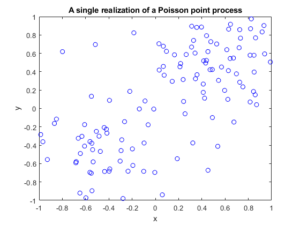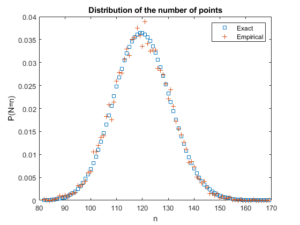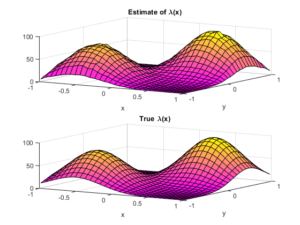##### Python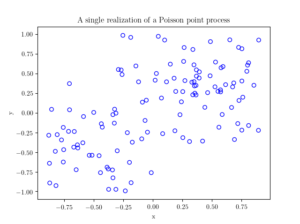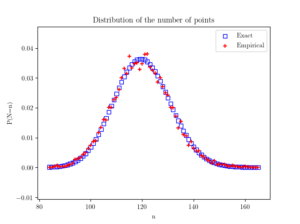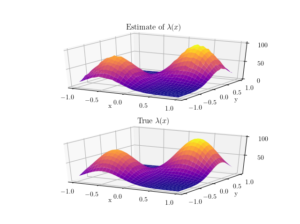I have not covered much new theoretical stuff in this post, so looking at the references in previous posts, such as this one, should help.

For two-dimensional histograms, I recommend going to the respective MATLAB and Python function websites.  Here’s an example of a two-dimensional histogram implemented in Python.

Probably the most difficult part for me was performing the plotting in Python.  I recommend these links:

https://plot.ly/python/3d-surface-plots/

https://chrisalbon.com/python/basics/set_the_color_of_a_matplotlib/

https://matplotlib.org/examples/color/colormaps_reference.html

## Code

All code from my posts, as always, can be found on the my GitHub repository. The code for this post is located here.

The estimating the statistical moments is standard. Performing the histograms is also routine, but when normalizing, you have to choose the option that returns the empirical estimate of the probability density function (pdf).

Fortunately, scientific programming languages usually have functions for performing two-dimensional histograms. What is  a bit tricky is how to normalize or rescale the bin counts. The histogram functions can,  for example, divide by the number of simulations, the area of each bin, or both. In the end, I chose the pdf option  in both MATLAB and Python to give an empirical estimate of the probability density function, and then multiplied it by the average number of points, which was calculated in the previous check. (Although,  I could have done this in a single step in MATLAB, but not in Python, so I chose to do it in a couple of steps in both languages so the code matches more closely.)

##### MATLAB

I used the surf function to plot the intensity function and its estimate; see below for details on the histograms.

close all;

%Simulation window parameters
xMin=-1;xMax=1;
yMin=-1;yMax=1;
xDelta=xMax-xMin;yDelta=yMax-yMin; %rectangle dimensions
areaTotal=xDelta*yDelta; %area of rectangle

numbSim=10^4; %number of simulations

s=0.5; %scale parameter

%Point process parameters
fun_lambda=@(x,y)(100*exp(-((x).^2+(y).^2)/s^2));%intensity function

%%%START -- find maximum lambda -- START %%%
%For an intensity function lambda, given by function fun_lambda,
%finds the maximum of lambda in a rectangular region given by
%[xMin,xMax,yMin,yMax].
funNeg=@(x)(-fun_lambda(x(1),x(2))); %negative of lambda
%initial value(ie centre)
xy0=[(xMin+xMax)/2,(yMin+yMax)/2];%initial value(ie centre)
%Set up optimization step
options=optimoptions('fmincon','Display','off');
%Find largest lambda value
[~,lambdaNegMin]=fmincon(funNeg,xy0,[],[],[],[],...
[xMin,yMin],[xMax,yMax],'',options);
lambdaMax=-lambdaNegMin;
%%%END -- find maximum lambda -- END%%%

%define thinning probability function
fun_p=@(x,y)(fun_lambda(x,y)/lambdaMax);

%for collecting statistics -- set numbSim=1 for one simulation
numbPointsRetained=zeros(numbSim,1); %vector to record number of points
for ii=1:numbSim
%Simulate Poisson point process
numbPoints=poissrnd(areaTotal*lambdaMax);%Poisson number of points
xx=xDelta*(rand(numbPoints,1))+xMin;%x coordinates of Poisson points
yy=xDelta*(rand(numbPoints,1))+yMin;%y coordinates of Poisson points

%calculate spatially-dependent thinning probabilities
p=fun_p(xx,yy);

%Generate Bernoulli variables (ie coin flips) for thinning
booleRetained=rand(numbPoints,1)<p; %points to be retained %x/y locations of retained points xxRetained=xx(booleRetained); yyRetained=yy(booleRetained); %collect number of points simulated numbPointsRetained(ii)=length(xxRetained); end %Plotting plot(xxRetained,yyRetained,'bo'); %plot retained points xlabel('x');ylabel('y'); %run empirical test on number of points generated if numbSim&gt;=10
%total mean measure (average number of points)
LambdaNumerical=integral2(fun_lambda,xMin,xMax,yMin,yMax)
%Test: as numbSim increases, numbPointsMean converges to LambdaNumerical
numbPointsMean=mean(numbPointsRetained)
%Test: as numbSim increases, numbPointsVar converges to LambdaNumerical
numbPointsVar=var(numbPointsRetained)

end


For the histogram section, I used the histcounts and histcounts2 functions respectively to estimate the distribution of the number of points and the intensity function. I used the pdf option.

Number of points

histcounts(numbPointsRetained,binEdges,'Normalization','pdf');


Locations of points

histcounts2(xxVectorAll,yyVectorAll,numbBins,'Normalization','pdf');

##### Python

I used the Matplotlib library to plot the intensity function and its estimate; see below for details on the histograms.

import numpy as np; #NumPy package for arrays, random number generation, etc
import matplotlib.pyplot as plt #for plotting
from matplotlib import cm #for heatmap plotting
from mpl_toolkits import mplot3d #for 3-D plots
from scipy.optimize import minimize #for optimizing
from scipy import integrate #for integrating
from scipy.stats import poisson #for the Poisson probability mass function

plt.close("all"); #close all plots

#Simulation window parameters
xMin=-1;xMax=1;
yMin=-1;yMax=1;
xDelta=xMax-xMin;yDelta=yMax-yMin; #rectangle dimensions
areaTotal=xDelta*yDelta;

numbSim=10**4; #number of simulations
numbBins=30; #number of bins for histogram

#Point process parameters
s=0.5; #scale parameter

def fun_lambda(x,y):
#intensity function
lambdaValue=80*np.exp(-((x+0.5)**2+(y+0.5)**2)/s**2)+100*np.exp(-((x-0.5)**2+(y-0.5)**2)/s**2);
return lambdaValue;

###START -- find maximum lambda -- START ###
#For an intensity function lambda, given by function fun_lambda,
#finds the maximum of lambda in a rectangular region given by
#[xMin,xMax,yMin,yMax].
def fun_Neg(x):
return -fun_lambda(x,x); #negative of lambda

xy0=[(xMin+xMax)/2,(yMin+yMax)/2];#initial value(ie centre)
#Find largest lambda value
resultsOpt=minimize(fun_Neg,xy0,bounds=((xMin, xMax), (yMin, yMax)));
lambdaNegMin=resultsOpt.fun; #retrieve minimum value found by minimize
lambdaMax=-lambdaNegMin;
###END -- find maximum lambda -- END ###

#define thinning probability function
def fun_p(x,y):
return fun_lambda(x,y)/lambdaMax;

#for collecting statistics -- set numbSim=1 for one simulation
numbPointsRetained=np.zeros(numbSim); #vector to record number of points
xxAll=[]; yyAll=[];

### START -- Simulation section -- START ###
for ii in range(numbSim):
#Simulate a Poisson point process
numbPoints = np.random.poisson(lambdaMax*areaTotal);#Poisson number of points
xx = xDelta*np.random.uniform(0,1,numbPoints)+xMin;#x coordinates of Poisson points
yy = yDelta*np.random.uniform(0,1,numbPoints)+yMin;#y coordinates of Poisson points

#calculate spatially-dependent thinning probabilities
p=fun_p(xx,yy);

#Generate Bernoulli variables (ie coin flips) for thinning
booleRetained=np.random.uniform(0,1,numbPoints)<p; #points to be thinned

#x/y locations of retained points
xxRetained=xx[booleRetained]; yyRetained=yy[booleRetained];
numbPointsRetained[ii]=xxRetained.size;
xxAll.extend(xxRetained); yyAll.extend(yyRetained);
### END -- Simulation section -- END ###

#Plotting a simulation
fig1 = plt.figure();
plt.scatter(xxRetained,yyRetained, edgecolor='b', facecolor='none');
plt.xlabel("x"); plt.ylabel("y");
plt.title('A single realization of a Poisson point process');
plt.show();

#run empirical test on number of points generated
###START -- Checking number of points -- START###
#total mean measure (average number of points)
#Test: as numbSim increases, numbPointsMean converges to LambdaNumerical
numbPointsMean=np.mean(numbPointsRetained);
#Test: as numbSim increases, numbPointsVar converges to LambdaNumerical
numbPointsVar=np.var(numbPointsRetained);
binEdges=np.arange(numbPointsRetained.min(),(numbPointsRetained.max()+1))-0.5;
pdfEmp, binEdges=np.histogram(numbPointsRetained, bins=binEdges,density=True);

nValues=np.arange(numbPointsRetained.min(),numbPointsRetained.max());
#analytic solution of probability density
pdfExact=(poisson.pmf(nValues,LambdaNumerical));

#Plotting
fig2 = plt.figure();
plt.scatter(nValues,pdfExact, color='b', marker='s',facecolor='none',label='Exact');
plt.scatter(nValues,pdfEmp, color='r', marker='+',label='Empirical');
plt.xlabel("n"); plt.ylabel("P(N=n)");
plt.title('Distribution of the number of points');
plt.legend();
plt.show();
###END -- Checking number of points -- END###

###START -- Checking locations -- START###
#2-D Histogram section
p_Estimate, xxEdges, yyEdges = np.histogram2d(xxAll, yyAll,bins=numbBins,density=True);
lambda_Estimate=p_Estimate*numbPointsMean;

xxValues=(xxEdges[1:]+xxEdges[0:xxEdges.size-1])/2;
yyValues=(yyEdges[1:]+yyEdges[0:yyEdges.size-1])/2;
X, Y = np.meshgrid(xxValues,yyValues) #create x/y matrices for plotting

#analytic solution of probability density
lambda_Exact=fun_lambda(X,Y);

#Plot empirical estimate
fig3 = plt.figure();
plt.rc('text', usetex=True);
plt.rc('font', family='serif');
ax=plt.subplot(211,projection='3d');
surf = ax.plot_surface(X, Y,lambda_Estimate,cmap=plt.cm.plasma);
plt.xlabel("x"); plt.ylabel("y");
plt.title('Estimate of $\lambda(x)$');
plt.locator_params(axis='x', nbins=5);
plt.locator_params(axis='y', nbins=5);
plt.locator_params(axis='z', nbins=3);
#Plot exact expression
ax=plt.subplot(212,projection='3d');
surf = ax.plot_surface(X, Y,lambda_Exact,cmap=plt.cm.plasma);
plt.xlabel("x"); plt.ylabel("y");
plt.title('True $\lambda(x)$');
plt.locator_params(axis='x', nbins=5);
plt.locator_params(axis='y', nbins=5);
plt.locator_params(axis='z', nbins=3);
###END -- Checking locations -- END###


For the histogram section, I used the histogram and histogram2d functions respectively to estimate the distribution of the number of points and the intensity function. I used the pdf option. (The SciPy website recommends not using the the normed option.)

Number of points

np.histogram(numbPointsRetained, bins=binEdges,density=True);


Locations of points

 np.histogram2d(xxArrayAll, yyArrayAll,bins=numbBins,density=True);


## Simulating an inhomogeneous Poisson point process

In previous posts I described how to simulate homogeneous Poisson point processes on a rectangledisk and triangle. But here I will simulate an inhomogeneous or nonhomogeneous Poisson point process. (Both of these terms are used, where the latter is probably more popular, but I prefer the former.) For such a point process, the points are not uniformly located on the underlying mathematical space on which the Poisson process is defined. This means that certain regions  will, on average, tend to have more (or less) points than other regions of the underlying space.

## Basics

Any Poisson point process is defined with a non-negative measure called the intensity or mean measure. I make the standard assumption that the intensity measure $$\Lambda$$ has a derivative $$\lambda(x,y)$$.  (I usually write a single $$x$$ to denote a point on the plane, that is $$x\in \mathbb{R}^2$$, but in this post I will write the $$x$$ and $$y$$ and coordinates separately.) The function $$\lambda(x,y)$$ is often called the intensity function or just intensity, which I further assume is bounded, so $$\lambda(x,y)<\infty$$ for all points in a simulation window $$W$$. Finally, I assume that the simulation window $$W$$ is a rectangle, but later I describe how to lift that assumption.

## Number of points

To simulate a point process,  the number of points and the point locations  in the simulation window $$W$$ are needed.  For any Poisson point process, the number of points is a Poisson random variable with a parameter (that is, a mean) $$\Lambda(W)$$, which under our previous assumptions is given by the integral

$$\Lambda(W)=\int_W \lambda(x,y)dxdy.$$

Assuming we can evaluate such an integral analytically or numerically, then the number of points is clearly not difficult to simulate.

## Locations of points

The difficulty lies in randomly positioning the points. But a defining property of the Poisson point process is its independence, which allows us to treat each point completely independently.  Positioning each point then comes down to suitably simulating two (or more) random variables for Poisson  point processes in two (or higher) dimensions.  Similarly, the standard methods used for simulating continuous random variables can be applied to simulating random point locations of a Poisson point process.

In theory, you can rescale the intensity function with the total measure of the simulation window, giving

$$f(x,y):=\frac{\lambda(x,y)}{\Lambda(W)}.$$

We can then interpret this rescaled intensity function $$f(x,y)$$  as the joint probability density of two random variables $$X$$ and $$Y$$, because it integrates to one,

$$\int_W f(x,y)dxdy=1.$$

Clearly the method for simulating an inhomogeneous Poisson point process depends on the nature of the intensity function. For the inhomogeneous case, the random variables $$X$$ and $$Y$$ are, in general, not independent.

### Transformation

To simulate an inhomogeneous Poisson point process, one method is to first simulate a homogeneous one, and then suitably transform the points according to deterministic function. For simple random variables, this transformation method is quick and easy to implement, if we can invert the probability distribution. For example, a uniform random variable $$U$$ defined on the interval $$(0,1)$$ can be used to give an exponential random variable by applying the transformation $$h(u)= -(1/\lambda)\log(u)$$, where $$\lambda>0$$, meaning $$h(U)$$ is an exponential random variable with parameter $$\lambda>0$$ (or mean $$1/\lambda$$).

Similarly for Poisson point processes, the transformation approach is fairly straightforward in a one-dimensional setting, but generally doesn’t work easily in two (or higher) dimensions. The reason being that often we cannot simulate the random variables $$X$$ and $$Y$$ independently, which means, in practice, we need first to simulate one random variable, then the other.

It is a bit easier if we can re-write the rescaled intensity function or joint probability density $$f(x,y)$$ as a product of single-variable functions $$f_X(x)$$ and $$f_Y(y)$$, meaning the random variables $$X$$ and $$Y$$ are independent. We can then simulate independently the random variables $$X$$ and $$Y$$, corresponding to the $$x$$ and $$y$$ coordinates of the points. But this would still require integrating and inverting the functions.

### Markov chain Monte Carlo

A now standard way to simulate jointly distributed random variables is to use  Markov chain Monte Carlo (MCMC), which we can also use to simulate the the $$X$$ and $$Y$$ random variables. Applying MCMC methods is simply applying random  point process operations repeatedly to all the points. But this is a bit too tricky and involved. Instead I’ll use a general yet simpler method based on thinning.

### Thinning

The thinning method is the arguably the simplest and most general way to simulate an inhomogeneous Poisson point process. If you’re unfamiliar with thinning, I recommend my previous post on thinning and the material I cite.

This simulation method is simply a type of acceptance-rejection method for simulating random variables. More specifically, it is the acceptance-rejection or rejection method, attributed to the great John von Neumann, for simulating a continuous random variable, say $$X$$, with some known probability density $$f(x)$$. The method accepts/retains or rejects/thins the outcome of each random variable/point depending on the outcome of a uniform random variable associated with each random variable/point.

The thinning or acceptance-rejection method is also appealing because it is an example of a perfect simulation method, which means the distribution of the simulated random variables or points will not be an approximation. This can be contrasted with typical MCMC methods, which, in theory, reach the desired distribution of the random variables in infinite time, which is clearly not possible in practice.

##### Simulating the homogeneous Poisson point process

First simulate a homogeneous Poisson point process with intensity value $$\lambda^*$$, which is an upper bound of the intensity function $$\lambda(x,y)$$. The simulation step is the easy part, but what value is $$\lambda^*$$?

I will use the maximum value that the intensity function $$\lambda(x,y)$$ takes, which I denote by

$$\lambda_{\max}:=\max_{(x,y)\in W}\lambda(x,y),$$

so I set $$\lambda^*=\lambda_{\max}$$. Of course with $$\lambda^*$$ being an upper bound, you can use any larger $$\lambda$$-value, so $$\lambda^*\geq \lambda_{\max}$$, but that just means more points will need to be thinned.

Scientific programming languages  have implemented algorithms that find or estimate minima of mathematical functions, meaning such an algorithm just needs to find the $$(x,y)$$ point that gives the minimum value of $$-\lambda(x,y)$$, which corresponds to the maximum value of $$\lambda(x,y)$$. What is very important is that the minimization procedure can handle constraints on the $$x$$ and $$y$$ values, which in our case of a rectangular simulation window $$W$$ are sometimes called box constraints.

##### Thinning the Poisson point process

All we need to do now is to thin the homogeneous Poisson point process with the thinning probability function

$$1-p(x,y)=\frac{\lambda(x,y)}{\lambda^*}.$$

This will randomly remove the points  so the remaining points will form a inhomogeneous Poisson point process with intensity function
$$(1-p(x,y))\lambda^* =\lambda(x).$$
As a result, we can see that provided $$\lambda^*\geq \lambda_{\max}>0$$, this procedure will give the right intensity function $$\lambda(x,y)$$.  I’ll skip the details on the point process still being Poisson after thinning, as I have already covered this in the thinning post.

## Empirical check

You can run an empirical check by simulating the point process a large number (say $$10^3$$ or $$10^4$$) of times, and collect statistics on the number of points. As the number of simulations increases, the average number of points should converge to the intensity measure $$\Lambda(W)$$, which is given by (perhaps numerically) evaluating the integral

$$\Lambda(W)=\int_W \lambda(x,y)dxdy.$$

This is a test for the intensity measure, a type of first moment, which will work for the intensity measure of any point process. But for Poisson point processes, the variance of the number of points will also converge to intensity measure $$\Lambda(W)$$, giving a second empirical test based on second moments.

An even more thorough test would be estimating an empirical distribution (that is, performing and normalizing a histogram) on the number of points. These checks will validate the number of points, but not the positioning of the points. In my next post I’ll cover how to perform these tests.

## Results

The homogeneous Poisson point process with intensity function $$\lambda(x)=100\exp(-(x^2+y^2)/s^2)$$, where $$s=0.5$$.  The results look similar to those in the thinning post, where the thinned points (that is, red circles) are generated from the same Poisson point process as the one that I have presented here.

MATLAB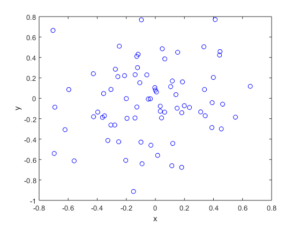Python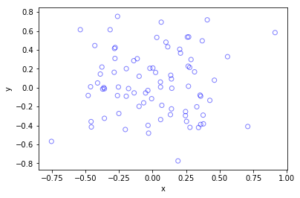## Method extensions

We can extend the thinning method for simulating inhomogeneous Poisson point processes a couple different ways.

### Using an inhomogeneous Poisson point process

The thinning method does not need to be applied to a homogeneous Poisson point process with intensity $$\lambda^*$$. In theory,  we could have simulated a suitably inhomogeneous Poisson point process with intensity function $$\lambda^*(x,y)$$, which has the condition

$$\lambda^*(x,y)\geq \lambda(x,y), \quad \forall (x,y)\in W .$$

Then this Poisson point process is thinned. But then we would still need to simulate the underlying Poisson point process, which often would be as difficult to simulate.

### Partitioning the simulation window

Perhaps the intensity of the Poisson point process only takes two values, $$\lambda_1$$ and $$\lambda_2$$, and the simulation window $$W$$ can be nicely divided or partitioned into two disjoints sets $$B_1$$ and $$B_2$$ (that is, $$B_1\cap B_2=\emptyset$$ and $$B_1\cup B_2=W$$), corresponding to the subregions of the two different intensity values.  The Poisson independence property allows us to simulate two independent Poisson point processes on the two subregions.

This approach only works for a piecewise constant intensity function. But if if the intensity function $$\lambda(x)$$ varies wildly, the simulation window can be partitioned into subregions $$B_1\dots,B_m$$ for different ranges of the intensity function $$\lambda(x)$$.  This allows us to simulate independent homogeneous Poisson point processes with different densities $$\lambda^*_1\dots, \lambda^*_m$$, where for each subregion $$B_i$$ we set

$$\lambda^*_i:=\max_{x\in B_i}\lambda(x,y).$$

The resulting Poisson point processes are then suitably thinned, resulting in a more efficient simulation method. (Although I imagine the gain would often be small.)

### Non-rectangular simulation windows

If you want to simulate on non-rectangular regions, which is not a disk or triangle, then the easiest way is to simulate a Poisson point process on a rectangle $$R$$ that completely covers the simulation window, so $$W \subset R\subset \mathbb{R}^2$$, and then set the intensity function $$\lambda$$ to zero for the region outside the simulation window $$W$$, that is $$\lambda (x,y)=0$$ when $$(x,y)\in R\setminus W$$.

In Section 2.5.2 of Stochastic Geometry and its Applications by Chiu, Stoyan, Kendall and Mecke, there is an outline of the thinning method that I used.  The same simulation section appears in the previous edition by Kendall and Mecke, though these books in general have little material on simulation methods.

More details on the thinning method and its connection to acceptance-rejection sampling are given in Section 2.3 of the applications-oriented book Poisson Point Processes by Streit. The acceptance-rejection method is covered in, for example, books on Monte Carlo methods, including Monte Carlo Strategies in Scientific Computing by Liu (in Section 2.2 )and Monte Carlo Methods in Financial Engineering by Glasserman (in Section 2.2.2).  This method and others for simulating generals random variable are covered in stochastic simulation books such as Uniform Random Variate Generation by Devroye and Stochastic Simulation: Algorithms and Analysis by Asmussen and Glynn.

Kroese and Botev have a good introduction to stochastic simulation in the edited collection Stochastic Geometry, Spatial Statistics and Random Fields : Models and Algorithms by Schmidt, where the relevant chapter (number 12) is also freely available online.  And of course there are lectures notes on the internet that cover simulation material.

## Code

All code from my posts, as always, can be found on the my GitHub repository. The code for this post is located here.  You can see that the code is very similar to that of the thinning code, which served as the foundation for this code. (Note how we now keep the points, so in the code the > has become  < on the line where the uniform variables are generated).

I have implemented the code in MATLAB and Python with an intensity function $$\lambda(x,y)=100\exp(-(x^2+y^2)/s^2)$$, where $$s>0$$ is a scale parameter.  Note that in the minimization step, the box constraints are expressed differently in MATLAB and Python: MATLAB first takes the minimum values then the maximum values, whereas Python first takes the $$x$$-values then the $$y$$-values.

The code presented here does not have the empirical check, which I described above, but it is implemented in the code located here.  For the parameters used in the code, the total measure is $$\Lambda(W)\approx 77.8068$$, meaning each simulation will generate on average almost seventy-eight points.

I have stopped writing code in R for a couple of reasons, but mostly because anything I could think of simulating in R can already be done in the spatial statistics library spatstat. I recommend the book Spatial Point Patterns, co-authored by the spatstat’s main contributor, Adrian Baddeley.

##### MATLAB

I have used the fmincon function to find the point that gives the minimum of $$-\lambda(x,y)$$.

%Simulation window parameters
xMin=-1;xMax=1;
yMin=-1;yMax=1;
xDelta=xMax-xMin;yDelta=yMax-yMin; %rectangle dimensions
areaTotal=xDelta*yDelta; %area of rectangle

s=0.5; %scale parameter

%Point process parameters
fun_lambda=@(x,y)(100*exp(-((x).^2+(y).^2)/s^2));%intensity function

%%%START -- find maximum lambda -- START %%%
%For an intensity function lambda, given by function fun_lambda,
%finds the maximum of lambda in a rectangular region given by
%[xMin,xMax,yMin,yMax].
funNeg=@(x)(-fun_lambda(x(1),x(2))); %negative of lambda
%initial value(ie centre)
xy0=[(xMin+xMax)/2,(yMin+yMax)/2];%initial value(ie centre)
%Set up optimization step
options=optimoptions('fmincon','Display','off');
%Find largest lambda value
[~,lambdaNegMin]=fmincon(funNeg,xy0,[],[],[],[],...
[xMin,yMin],[xMax,yMax],'',options);
lambdaMax=-lambdaNegMin;
%%%END -- find maximum lambda -- END%%%

%define thinning probability function
fun_p=@(x,y)(fun_lambda(x,y)/lambdaMax);

%Simulate Poisson point process
numbPoints=poissrnd(areaTotal*lambdaMax);%Poisson number of points
xx=xDelta*(rand(numbPoints,1))+xMin;%x coordinates of Poisson points
yy=xDelta*(rand(numbPoints,1))+yMin;%y coordinates of Poisson points

%calculate spatially-dependent thinning probabilities
p=fun_p(xx,yy);

%Generate Bernoulli variables (ie coin flips) for thinning
booleRetained=rand(numbPoints,1)<p; %points to be thinned

%x/y locations of retained points
xxRetained=xx(booleRetained); yyRetained=yy(booleRetained);

%Plotting
plot(xxRetained,yyRetained,'bo'); %plot retained points
xlabel('x');ylabel('y');


The box constraints for the optimization step were expressed as:

[xMin,yMin],[xMax,yMax]

##### Python

I have used the minimize function in SciPy.

import numpy as np; #NumPy package for arrays, random number generation, etc
import matplotlib.pyplot as plt #For plotting
from scipy.optimize import minimize #For optimizing
from scipy import integrate

#Simulation window parameters
xMin=-1;xMax=1;
yMin=-1;yMax=1;
xDelta=xMax-xMin;yDelta=yMax-yMin; #rectangle dimensions
areaTotal=xDelta*yDelta;

s=0.5; #scale parameter

#Point process parameters
def fun_lambda(x,y):
return 100*np.exp(-(x**2+y**2)/s**2); #intensity function

###START -- find maximum lambda -- START ###
#For an intensity function lambda, given by function fun_lambda,
#finds the maximum of lambda in a rectangular region given by
#[xMin,xMax,yMin,yMax].
def fun_Neg(x):
return -fun_lambda(x,x); #negative of lambda

xy0=[(xMin+xMax)/2,(yMin+yMax)/2];#initial value(ie centre)
#Find largest lambda value
resultsOpt=minimize(fun_Neg,xy0,bounds=((xMin, xMax), (yMin, yMax)));
lambdaNegMin=resultsOpt.fun; #retrieve minimum value found by minimize
lambdaMax=-lambdaNegMin;
###END -- find maximum lambda -- END ###

#define thinning probability function
def fun_p(x,y):
return fun_lambda(x,y)/lambdaMax;

#Simulate a Poisson point process
numbPoints = np.random.poisson(lambdaMax*areaTotal);#Poisson number of points
xx = np.random.uniform(0,xDelta,((numbPoints,1)))+xMin;#x coordinates of Poisson points
yy = np.random.uniform(0,yDelta,((numbPoints,1)))+yMin;#y coordinates of Poisson points

#calculate spatially-dependent thinning probabilities
p=fun_p(xx,yy);

#Generate Bernoulli variables (ie coin flips) for thinning
booleRetained=np.random.uniform(0,1,((numbPoints,1)))<p; #points to be thinned

#x/y locations of retained points
xxRetained=xx[booleRetained]; yyRetained=yy[booleRetained];

#Plotting
plt.scatter(xxRetained,yyRetained, edgecolor='b', facecolor='none', alpha=0.5 );
plt.xlabel("x"); plt.ylabel("y");
plt.show();


The box constraints  were expressed as:

(xMin, xMax), (yMin, yMax)

##### Julia

After writing this post, I later wrote the code in Julia. The code is here and my thoughts about Julia are here.

## Thinning point processes

One way to create new point processes is to apply thinning to a point process. As I mentioned in a previous post on point process operations, thinning is a random operation applied to the points of an underlying point process, where the points are thinned (or removed) or retained (or kept) according to some probabilistic rule. Both the thinned and retained points form two separate point processes, but one usually focuses on the retained points. Given an underlying point process, the complexity of the thinning rule will result in different types of point processes.

## Thinning types

Thinning can be statistically independent or dependent, meaning that the probability of thinning any point is either independent or dependent of thinning other points. The more tractable case is statistically independent thinning, which is the thinning type covered here. We can further group this thinning into two types based on whether the thinning rule depends on the locations of the point. (I use the word location, instead of point, to refer to where a point of a point process is located on the underlying mathematical space on which the point process is defined.)

##### Spatially independent thinning

The simplest thinning operation is one that does not depend on point locations. This thinning is sometimes referred to as $$p$$-thinning, where the constant $$p$$ has the condition $$0\leq p \leq 1$$ because it is the probability of thinning a single point. Simply put, the probability of a point being thinned does not depend on the point locations.

Example

We can liken the thinning action to flipping a biased coin with probability of $$p$$ for heads (or tails) for each point of the underlying point process, and then removing the point if a head (or tails) occurs. If there were a constant number $$n$$ of points of the underlying point process, then the number of thinned (or retained) points will form a binomial random variable with parameters $$n$$ and $$p$$ (or $$1-p$$).

Simulation

Simulating this thinning operation is rather straightforward. Given a realization of a point process, for each point a uniform random variable on the interval $$(0,1)$$, simply generate or simulate, and if this random variable is less than $$p$$, remove the point. In the code section below, I have shown how this thinning operation is implemented.

##### Spatially dependent thinning

To generalize the idea of $$p$$-thinning, we can simply require that the thinning probability of any point depends on its location $$x$$, which gives us the concept of $$p(x)$$-thinning. (I write a single $$x$$ to denote a point on the plane, that is $$x\in \mathbb{R}^2$$, instead of writing, for example, the $$x$$ and $$y$$ and coordinates separately.) More precisely, the probability of thinning a point is given by a function $$p(x)$$ such that $$0 \leq p(x)\leq 1$$, but all point thinnings occur independently of each other. In other words, this is a spatially dependent thinning that is statistically independent.

Example

I’ll illustrate the concept of spatially dependent thinning with a somewhat contrived example. We assume that the living locations of all the people in the world form a point process on a (slightly squashed) sphere. Let’s say that Earth has become overpopulated, particularly in the Northern Hemisphere, so we decide to randomly choose people and send them off to another galaxy, but we do it based on how far they live from the North Pole. The thinning rule is, for example, $$p(x)= \exp(- |x|^2/s^2)$$, where $$|x|$$ is the distance to the North Pole and $$s>0$$ is some constant for distance scaling.

Put another way, a person at location $$x$$ flips a biased coin with the probability of, say, heads being equal to $$p(x)=\exp(- |x|^2/s^2)$$. If a head comes up, then that person is removed from the planet. With the maximum of $$p(x)$$ is at the North Pole, we can see that the lucky (or unlucky?) people in countries like Australia, NZ, South Africa, Argentina and Chile, are more likely not to be sent off (that is, thinned). For people who live comparable distances from the North Pole, the removal probabilities are similar in value, yet the events of being remove remain independent. For example, the probabilities of removing any two people from Lesotho are similar in value, but these two random events are still completely independent of each other.

Simulation

Simulating a spatially dependent thinning is just slightly more involved than the spatially independent case. Given a realization of a point process, for each point at, say, $$x$$, simply generate or simulate a uniform random variable on the interval $$(0,1)$$, and if this random variable is less than $$p(x)$$, remove the point.

In the code section, I have shown how this thinning operation is implemented with an example like the above one, but on a rectangular region of Cartesian space. In this setting, the maximum of $$p(x)$$ is at the origin, resulting in more points being thinned in this region.

## Thinning a Poisson point process

Perhaps not surprisingly, under the thinning operation the Poisson point process exhibits a closure property, meaning that a Poisson point process thinned in a certain way gives another Poisson point process.  More precisely, if the thinning operation is statistically independent, then the resulting point process formed from the retained points is also a Poisson point process, regardless if it is spatially independent or dependent thinning. The resulting intensity (interpreted as the average density of points) of this new Poisson point process has a simple expression.

##### Homogeneous case

For a spatially independent $$p$$-thinning, if the original (or underlying) Poisson point process is homogeneous with intensity $$\lambda$$, then the point process formed from the retained points is a homogeneous Poisson point process with intensity $$\lambda$$.  (There are different ways to prove this, but you can gain some intuition behind the proof by conditioning on the Poisson number of points and then applying the total law of probability. Using generating functions helps.)

##### Inhomogeneous case

More generally, if we apply a spatially dependent $$p(x)$$-thinning to a  Poisson point process has a intensity $$\lambda$$, then the retained points form a  an inhomogeneous or nonhomogeneous Poisson point process with $$\lambda p(x)$$,  due to the spatial dependence in the thinning function $$p(x)$$. This gives a way to simulate such Poisson point processes, which I’ll cover in another post.

##### Splitting

We can see by symmetry that if we look at the thinned points, then the resulting point process is also a Poisson point process, but with intensity $$(1-p(x))\lambda$$. The retained and thinned points both form Poisson point processes, but what is really interesting is these two point processes are independent of each other.  This means that any random configuration that occurs among the retained points is independent of any configurations among the thinned points.

This ability to split a Poisson point processes into independent ones is sometimes called the splitting property.

## Possible applications

Thinning point processes has the immediate application of creating new point processes. It can also be used to randomly generate two point processes from one. In network applications, a simple example is using the thinning procedure to model random sleep schemes in wireless networks, where random subsets of the network have been powered down.

## Code

All code from my posts, as always, can be found on the my GitHub repository. The code for this post is located here.

##### Spatially independent thinning

I have implemented in code the simple $$p$$-thinning operation applied to a Poisson point process on a rectangle, but in theory any point process can be used for the underlying point process that is thinned.

MATLAB

%Simulation window parameters
xMin=-1;xMax=1;
yMin=-1;yMax=1;
xDelta=xMax-xMin;yDelta=yMax-yMin; %rectangle dimensions
areaTotal=xDelta*yDelta; %area of rectangle

%Point process parameters
lambda=100; %intensity (ie mean density) of the Poisson process

%Thinning probability parameters
sigma=1;
p=0.25; %thinning probability

%Simulate Poisson point process
numbPoints=poissrnd(areaTotal*lambda);%Poisson number of points
xx=xDelta*(rand(numbPoints,1))+xMin;%x coordinates of Poisson points
yy=xDelta*(rand(numbPoints,1))+yMin;%y coordinates of Poisson points

%Generate Bernoulli variables (ie coin flips) for thinning
booleThinned=rand(numbPoints,1)>p; %points to be thinned
booleRetained=~booleThinned; %points to be retained

%x/y locations of thinned points
xxThinned=xx(booleThinned); yyThinned=yy(booleThinned);
%x/y locations of retained points
xxRetained=xx(booleRetained); yyRetained=yy(booleRetained);

%Plotting
plot(xxRetained,yyRetained,'bo'); %plot retained points
hold on; plot(xxThinned,yyThinned,'ro'); %plot thinned points
xlabel('x');ylabel('y');


R

#Simulation window parameters
xMin=-1;xMax=1;
yMin=-1;yMax=1;
xDelta=xMax-xMin;yDelta=yMax-yMin; #rectangle dimensions
areaTotal=xDelta*yDelta;

#Point process parameters
lambda=100; #intensity (ie mean density) of the Poisson process

#Thinning probability
p=0.25;

#Simulate a Poisson point process
numbPoints=rpois(1,areaTotal*lambda);#Poisson number of points
xx=xDelta*runif(numbPoints)+xMin;#x coordinates of Poisson points
yy=xDelta*runif(numbPoints)+yMin;#y coordinates of Poisson points

#Generate Bernoulli variables (ie coin flips) for thinning
booleThinned=runif(numbPoints)>p; #points to be thinned
booleRetained=!booleThinned; #points to be retained

#x/y locations of thinned points
xxThinned=xx[booleThinned]; yyThinned=yy[booleThinned];
#x/y locations of retained points
xxRetained=xx[booleRetained]; yyRetained=yy[booleRetained];

#Plotting
par(pty="s")
plot(xxRetained,yyRetained,'p',xlab='x',ylab='y',col='blue'); #plot retained points
points(xxThinned,yyThinned,col='red'); #plot thinned points



Of course, as I have mentioned before, simulating a spatial point processes in R is even easier with the powerful spatial statistics library spatstat.  With this library, thinning can be done in quite a general way by using the function rthin.

Python

import numpy as np; #NumPy package for arrays, random number generation, etc
import matplotlib.pyplot as plt

#Simulation window parameters
xMin=-1;xMax=1;
yMin=-1;yMax=1;
xDelta=xMax-xMin;yDelta=yMax-yMin; #rectangle dimensions
areaTotal=xDelta*yDelta;

#Point process parameters
lambda0=100; #intensity (ie mean density) of the Poisson process

#Thinning probability
p=0.25;

#Simulate a Poisson point process
numbPoints = np.random.poisson(lambda0*areaTotal);#Poisson number of points
xx = np.random.uniform(0,xDelta,((numbPoints,1)))+xMin;#x coordinates of Poisson points
yy = np.random.uniform(0,yDelta,((numbPoints,1)))+yMin;#y coordinates of Poisson points

#Generate Bernoulli variables (ie coin flips) for thinning
booleThinned=np.random.uniform(0,1,((numbPoints,1)))>p; #points to be thinned
booleRetained=~booleThinned; #points to be retained

#x/y locations of thinned points
xxThinned=xx[booleThinned]; yyThinned=yy[booleThinned];
#x/y locations of retained points
xxRetained=xx[booleRetained]; yyRetained=yy[booleRetained];

#Plotting
plt.scatter(xxRetained,yyRetained, edgecolor='b', facecolor='none', alpha=0.5 );
plt.scatter(xxThinned,yyThinned, edgecolor='r', facecolor='none', alpha=0.5 );
plt.xlabel("x"); plt.ylabel("y");
plt.show();

##### Spatially dependent thinning

I have implemented in code a $$p(x)$$-thinning operation with the function $$p(x)=\exp(-|x|^2/s^2)$$, where $$|x|$$ is the Euclidean distance from $$x$$ to the origin. This small changes means that in the code there will be a vector or array of $$p$$ values instead of a single $$p$$ value in the section where the uniform random variables are generated and compared said $$p$$ values.  (Lines 24, 26 and 28 respectively in the MATLAB, R and Python code presented below.)

Again, I have applied thinning to a Poisson point process on a rectangle, but in theory any point process can be used for the underlying point process.

MATLAB

%Simulation window parameters
xMin=-1;xMax=1;
yMin=-1;yMax=1;
xDelta=xMax-xMin;yDelta=yMax-yMin; %rectangle dimensions
areaTotal=xDelta*yDelta; %area of rectangle

%Point process parameters
lambda=100; %intensity (ie mean density) of the Poisson process

%Thinning probability parameters
sigma=0.5; %scale parameter for thinning probability function
%define thinning probability function
fun_p=@(s,x,y)(exp(-(x.^2+y.^2)/s^2));

%Simulate Poisson point process
numbPoints=poissrnd(areaTotal*lambda);%Poisson number of points
xx=xDelta*(rand(numbPoints,1))+xMin;%x coordinates of Poisson points
yy=xDelta*(rand(numbPoints,1))+yMin;%y coordinates of Poisson points

%calculate spatially-dependent thinning probabilities
p=fun_p(sigma,xx,yy);

%Generate Bernoulli variables (ie coin flips) for thinning
booleThinned=rand(numbPoints,1)>p; %points to be thinned
booleRetained=~booleThinned; %points to be retained

%x/y locations of thinned points
xxThinned=xx(booleThinned); yyThinned=yy(booleThinned);
%x/y locations of retained points
xxRetained=xx(booleRetained); yyRetained=yy(booleRetained);

%Plotting
plot(xxRetained,yyRetained,'bo'); %plot retained points
hold on; plot(xxThinned,yyThinned,'ro'); %plot thinned points
xlabel('x');ylabel('y');


R

#Simulation window parameters
xMin=-1;xMax=1;
yMin=-1;yMax=1;
xDelta=xMax-xMin;yDelta=yMax-yMin; #rectangle dimensions
areaTotal=xDelta*yDelta;

#Point process parameters
lambda=100; #intensity (ie mean density) of the Poisson process

#Thinning probability parameters
sigma=0.5; #scale parameter for thinning probability function
#define thinning probability function
fun_p <- function(s,x,y) {
exp(-(x^2 + y^2)/s^2);
}

#Simulate a Poisson point process
numbPoints=rpois(1,areaTotal*lambda);#Poisson number of points
xx=xDelta*runif(numbPoints)+xMin;#x coordinates of Poisson points
yy=xDelta*runif(numbPoints)+yMin;#y coordinates of Poisson points

#calculate spatially-dependent thinning probabilities
p=fun_p(sigma,xx,yy);

#Generate Bernoulli variables (ie coin flips) for thinning
booleThinned=runif(numbPoints)<p; #points to be thinned
booleRetained=!booleThinned; #points to be retained

#x/y locations of thinned points
xxThinned=xx[booleThinned]; yyThinned=yy[booleThinned];
#x/y locations of retained points
xxRetained=xx[booleRetained]; yyRetained=yy[booleRetained];

#Plotting
par(pty="s")
plot(xxRetained,yyRetained,'p',xlab='x',ylab='y',col='blue'); #plot retained points
points(xxThinned,yyThinned,col='red'); #plot thinned points


Again, use the spatial statistics library spatstat, which has the function rthin.

Python

import numpy as np; #NumPy package for arrays, random number generation, etc
import matplotlib.pyplot as plt

#Simulation window parameters
xMin=-1;xMax=1;
yMin=-1;yMax=1;
xDelta=xMax-xMin;yDelta=yMax-yMin; #rectangle dimensions
areaTotal=xDelta*yDelta;

#Point process parameters
lambda0=100; #intensity (ie mean density) of the Poisson process

#Thinning probability parameters
sigma=0.5; #scale parameter for thinning probability function
#define thinning probability function
def fun_p(s, x, y):
return np.exp(-(x**2+y**2)/s**2);

#Simulate a Poisson point process
numbPoints = np.random.poisson(lambda0*areaTotal);#Poisson number of points
xx = np.random.uniform(0,xDelta,((numbPoints,1)))+xMin;#x coordinates of Poisson points
yy = np.random.uniform(0,yDelta,((numbPoints,1)))+yMin;#y coordinates of Poisson points

#calculate spatially-dependent thinning probabilities
p=fun_p(sigma,xx,yy);

#Generate Bernoulli variables (ie coin flips) for thinning
booleThinned=np.random.uniform(0,1,((numbPoints,1)))>p; #points to be thinned
booleRetained=~booleThinned; #points to be retained

#x/y locations of thinned points
xxThinned=xx[booleThinned]; yyThinned=yy[booleThinned];
#x/y locations of retained points
xxRetained=xx[booleRetained]; yyRetained=yy[booleRetained];

#Plotting
plt.scatter(xxRetained,yyRetained, edgecolor='b', facecolor='none', alpha=0.5 );
plt.scatter(xxThinned,yyThinned, edgecolor='r', facecolor='none', alpha=0.5 );
plt.xlabel("x"); plt.ylabel("y");
plt.show();


### Results

In the plotted results, the blue and red circles represent respectively the retained and thinned points.

##### Spatially independent thinning

For these results, I used a thinning probability $$p=0.25$$, which means that roughly a quater of the points will be thinned, so on average the ratio of blue to red circles is three to one.

MATLAB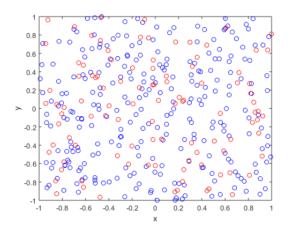R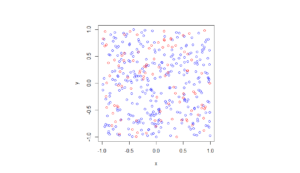Python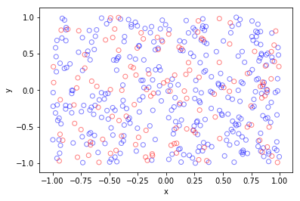##### Spatially dependent thinning

Observe how there are more thinned points (that is, red circles) near the origin, which is of course where the thinning function $$p(x)=\exp(-|x|^2/s^2)$$ attains its maximum.

MATLAB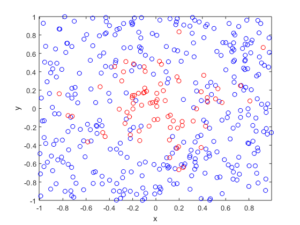R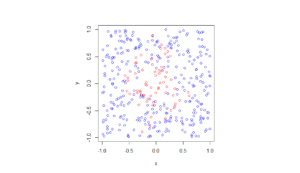Python